# Concise Selina Solutions for Class 9 Maths Chapter 1- Rational And Irrational Numbers

Selina solutions for Class 9 Maths Chapter 1 Rational And Irrational Numbers are provided here. Class 9 is an important phase of a student’s life, the concepts which are taught in Class 9 are vital to be understood as these concepts are continued in Class 10. To score good marks in Class 9 mathematics examination, it is advised to solve questions provided in each exercise of all the chapters in the Selina book. These Selina solutions for Class 9 Maths help the students in understanding all the concepts in a better way. Download pdf of Class 9 Maths Chapter 1 Selina Solutions from the given links.

### Download PDF of Selina Solutions for Class 9 Maths Chapter 1- Rational And Irrational Numbers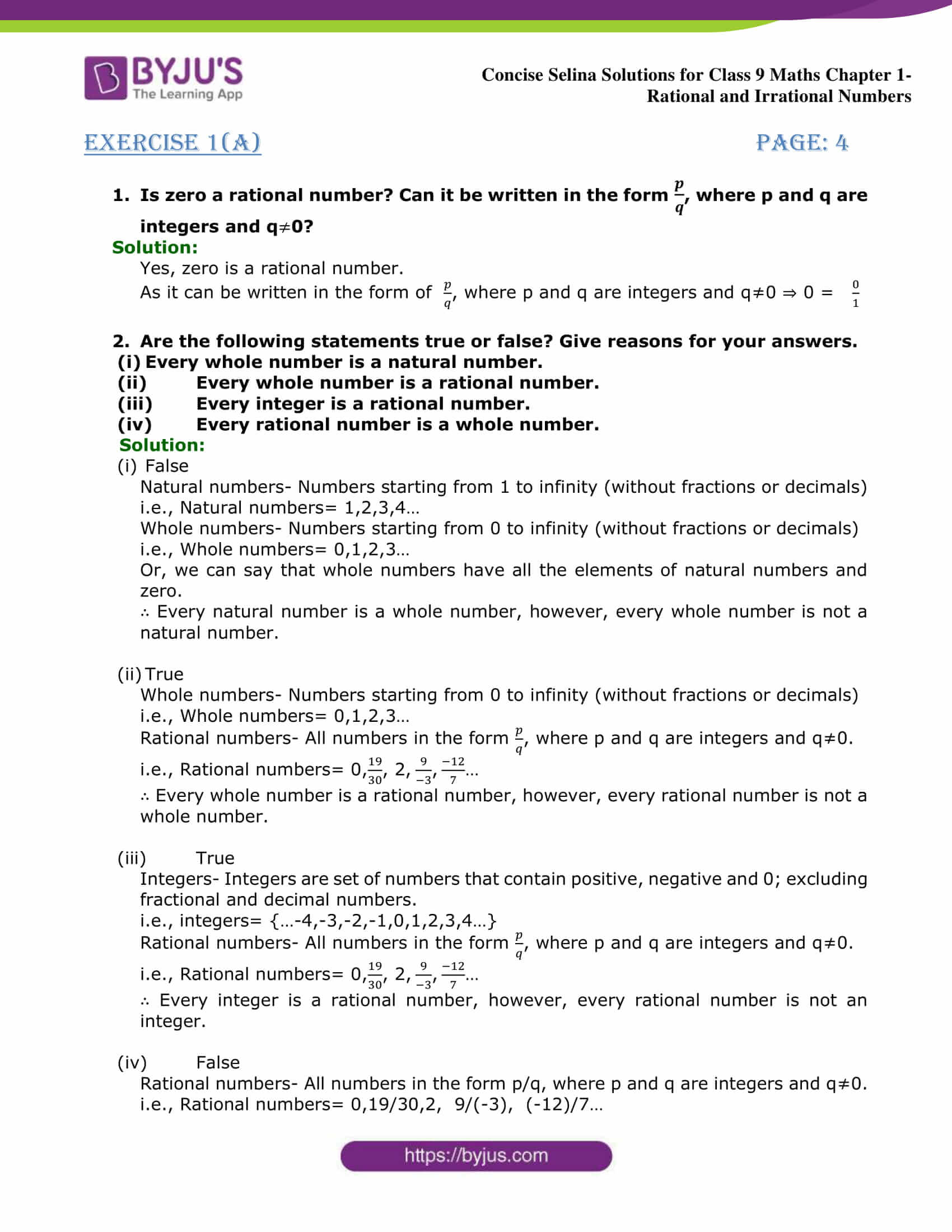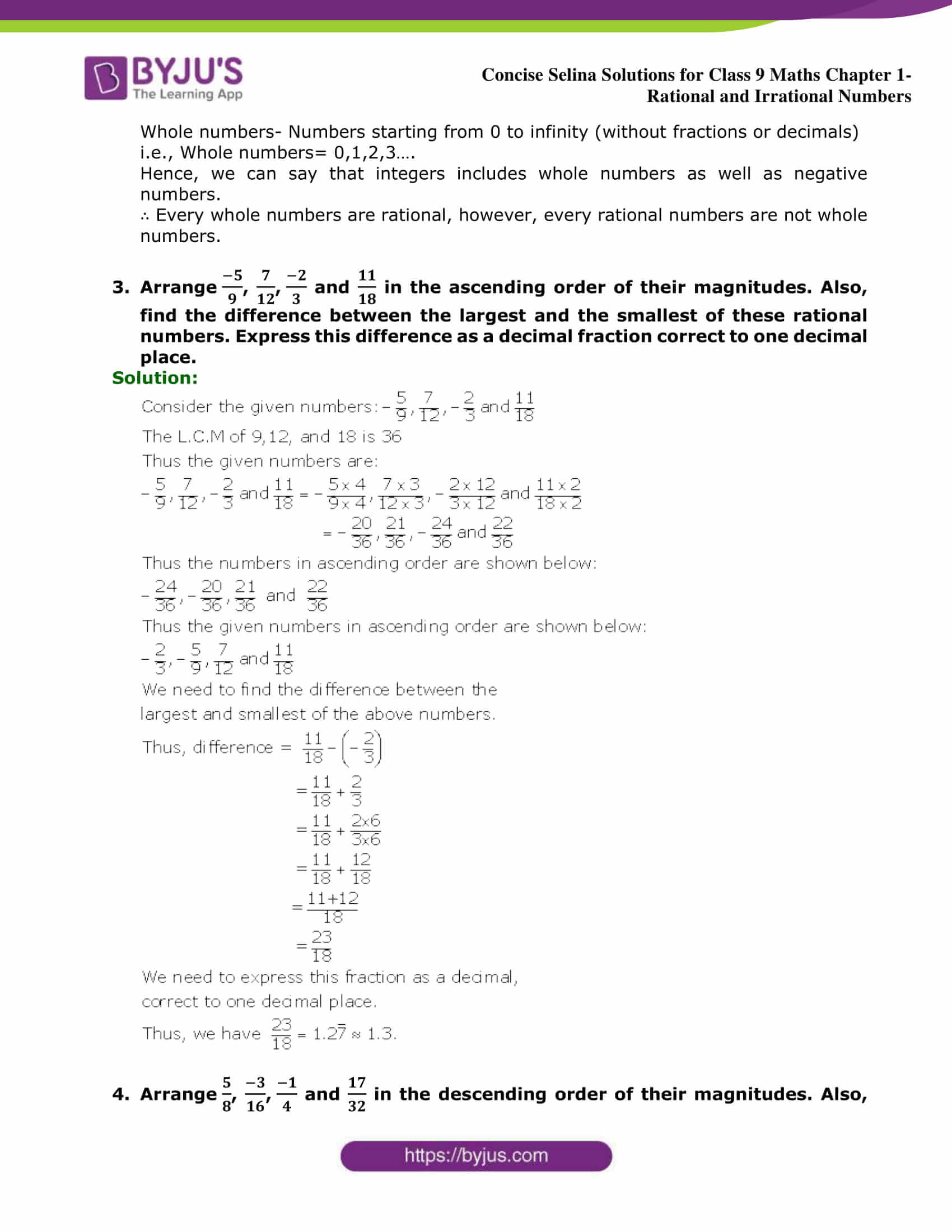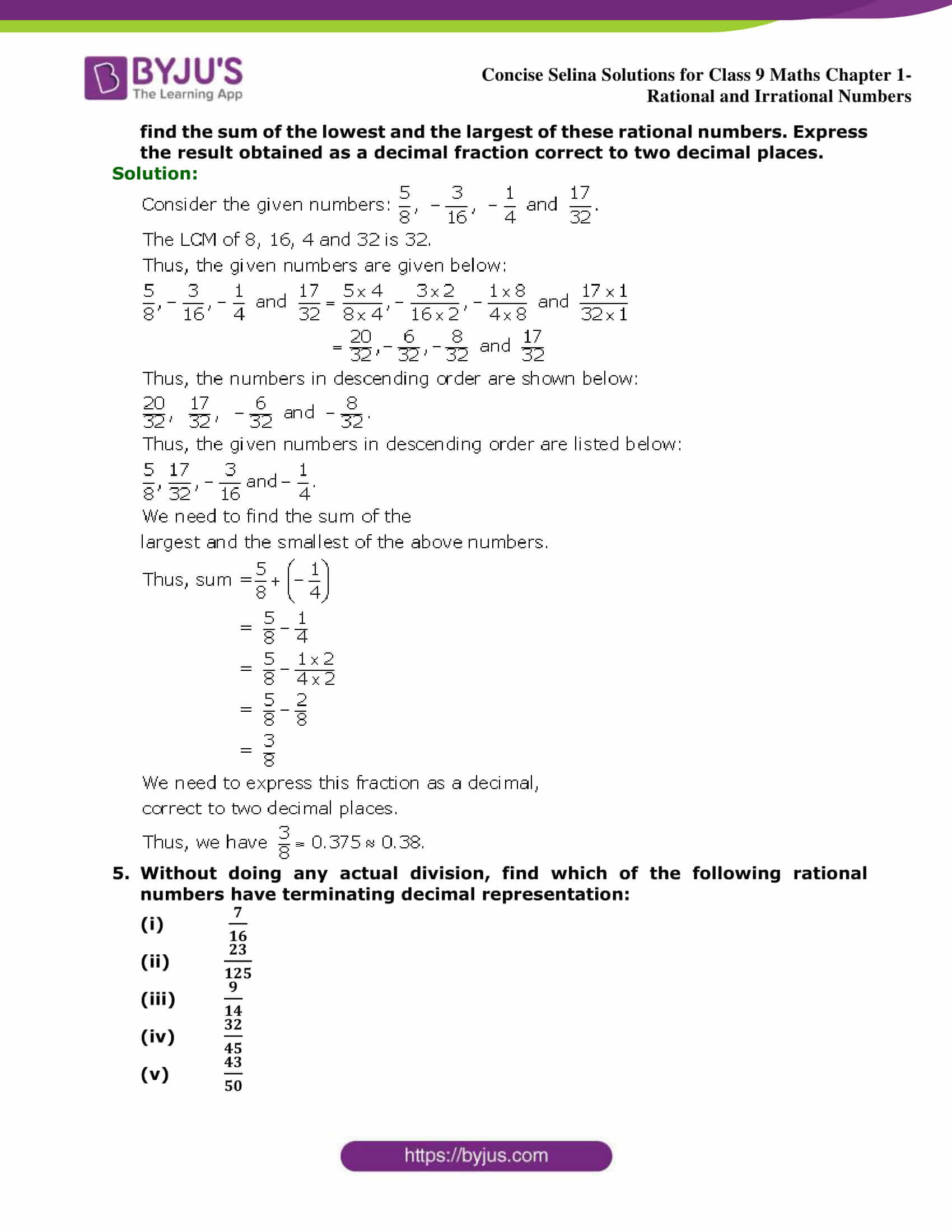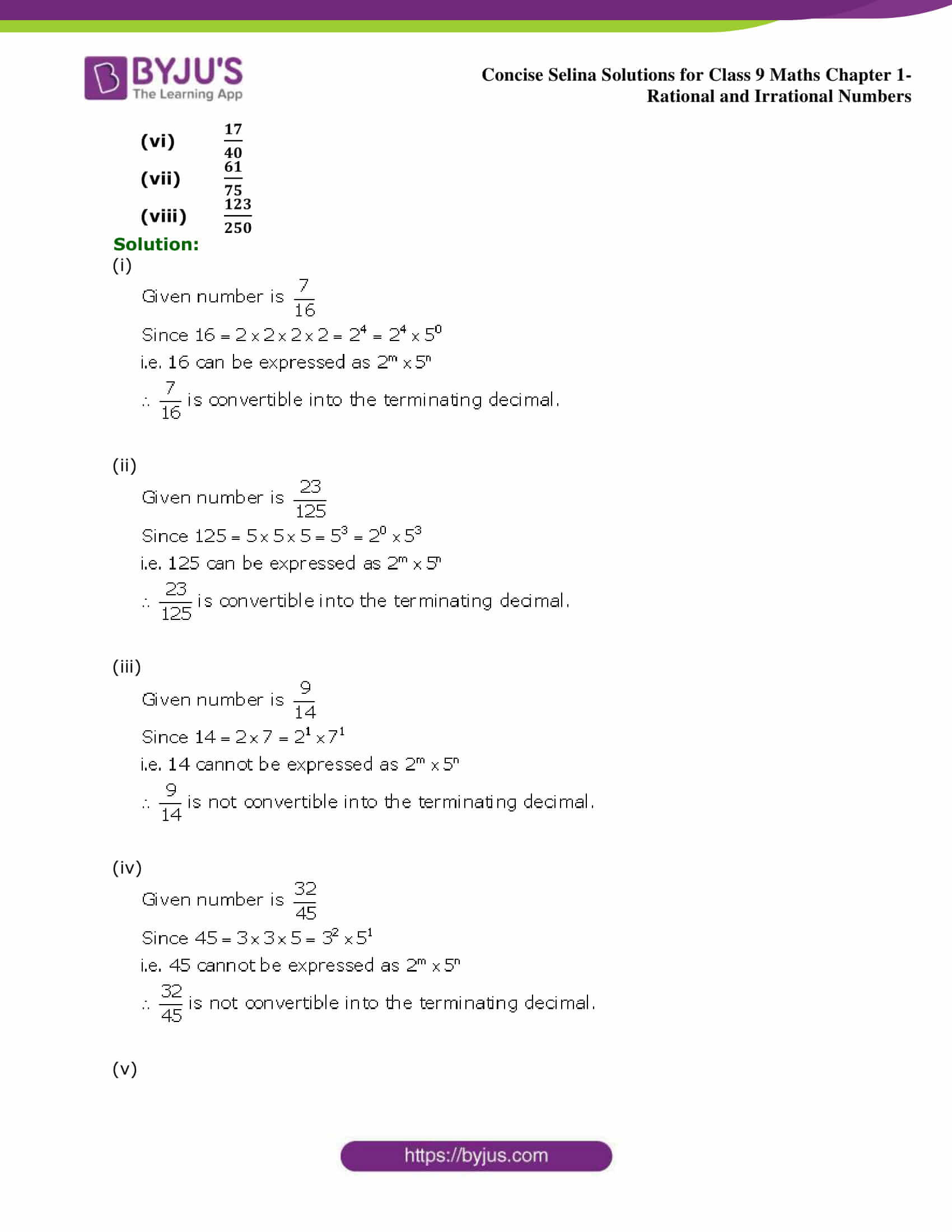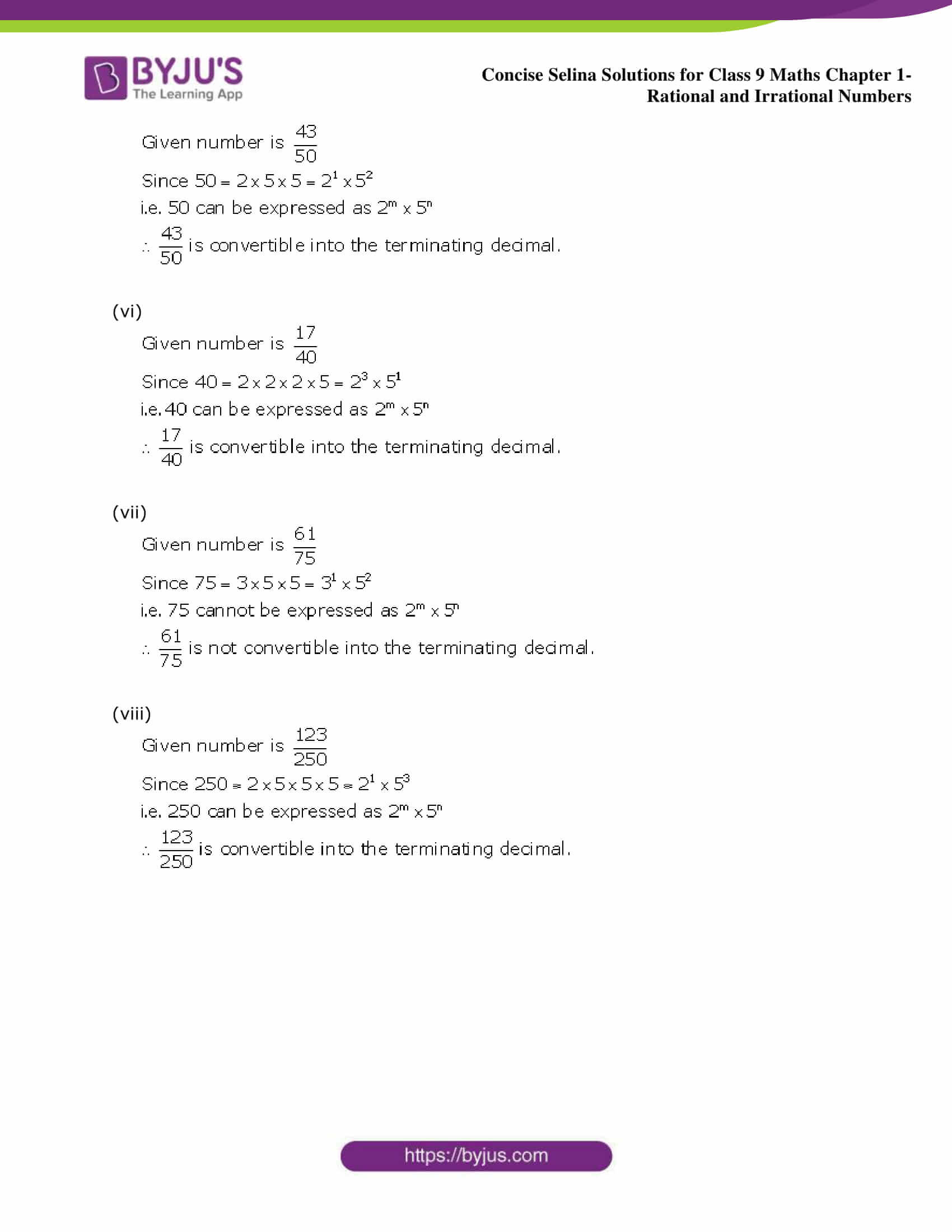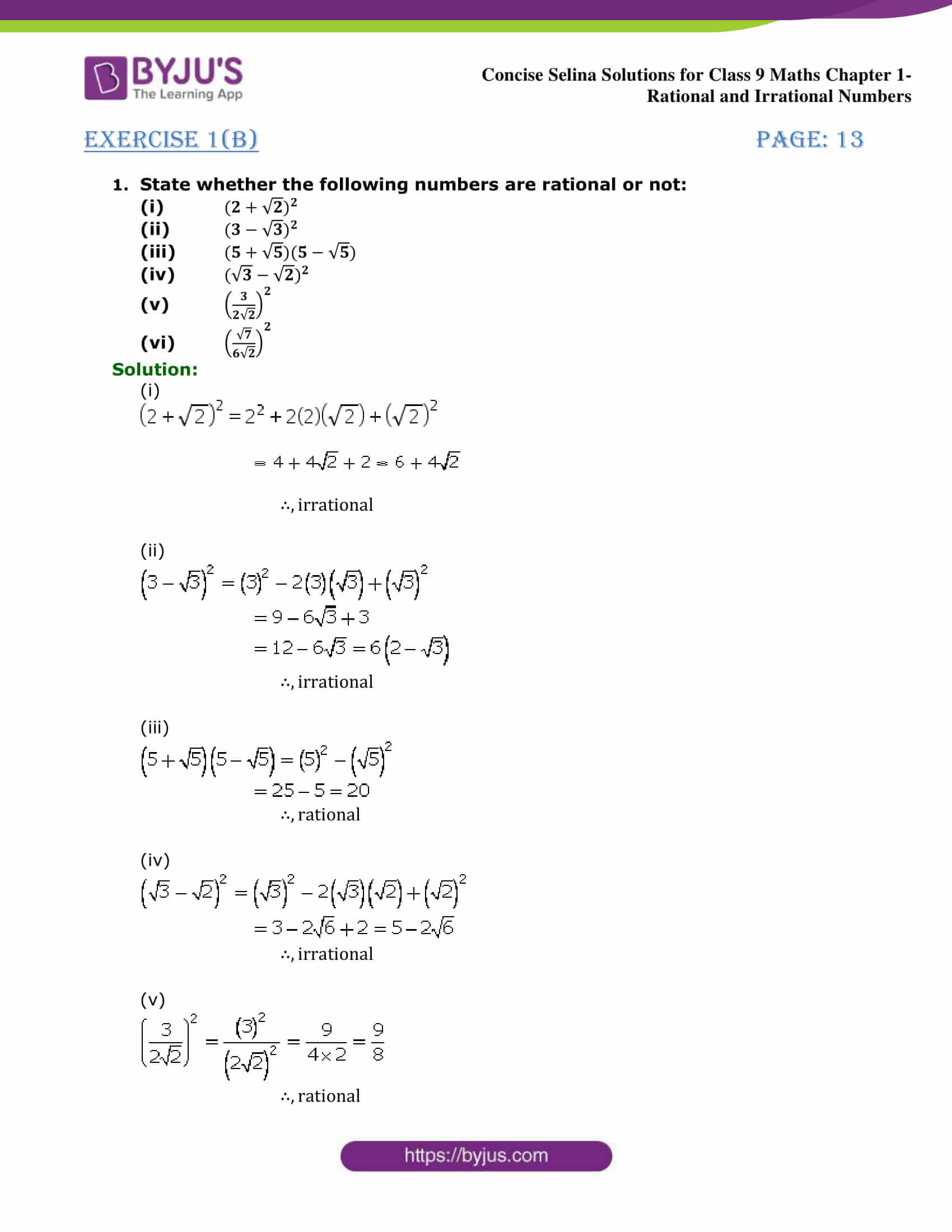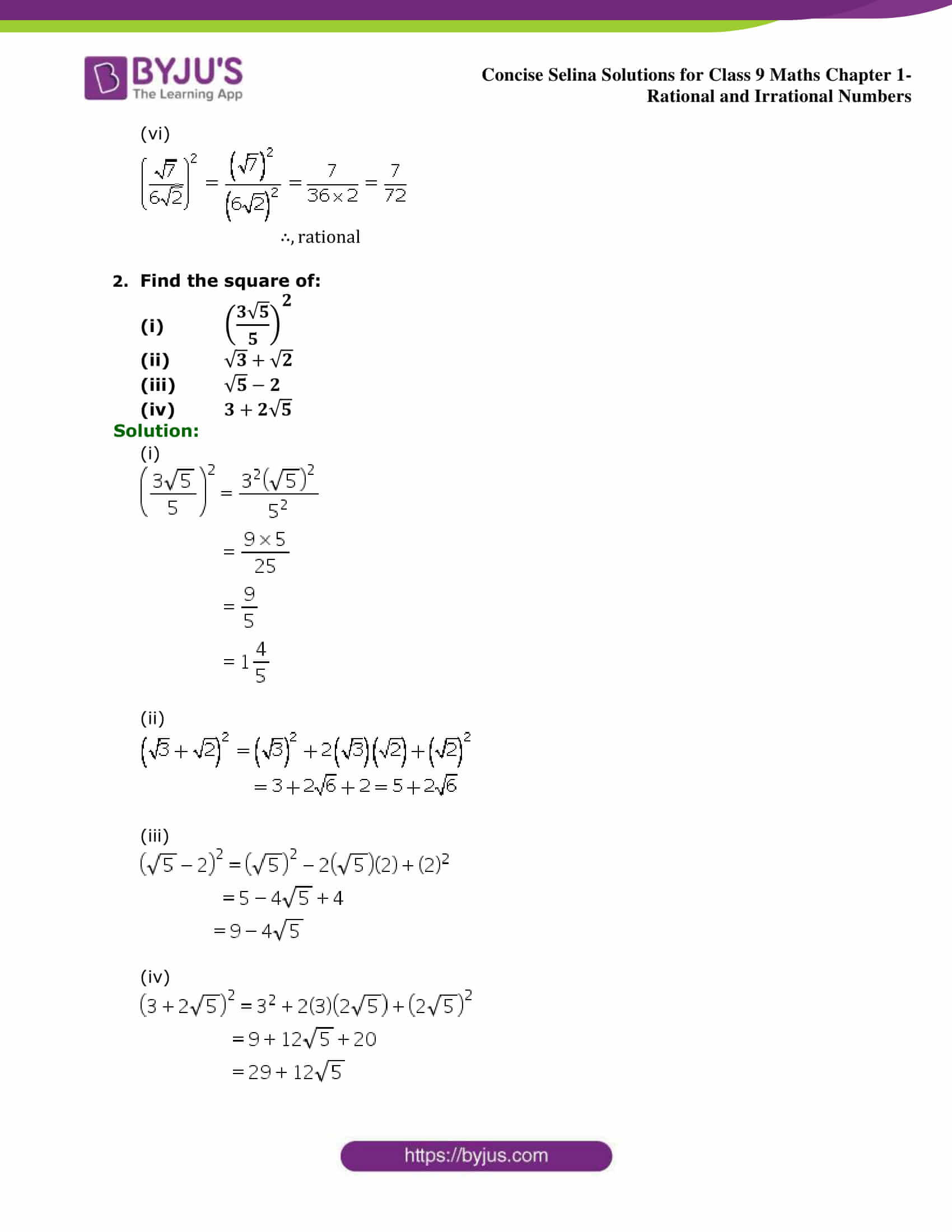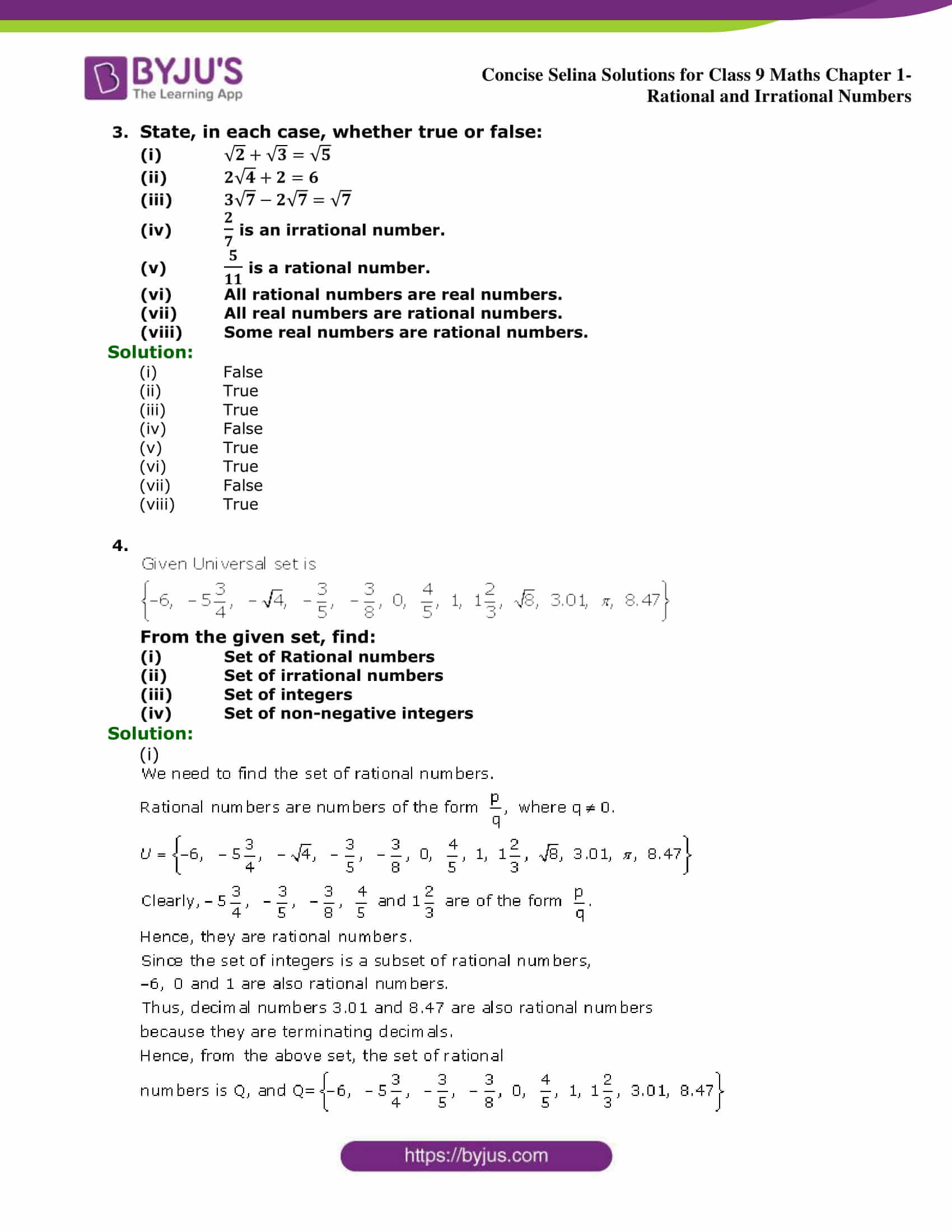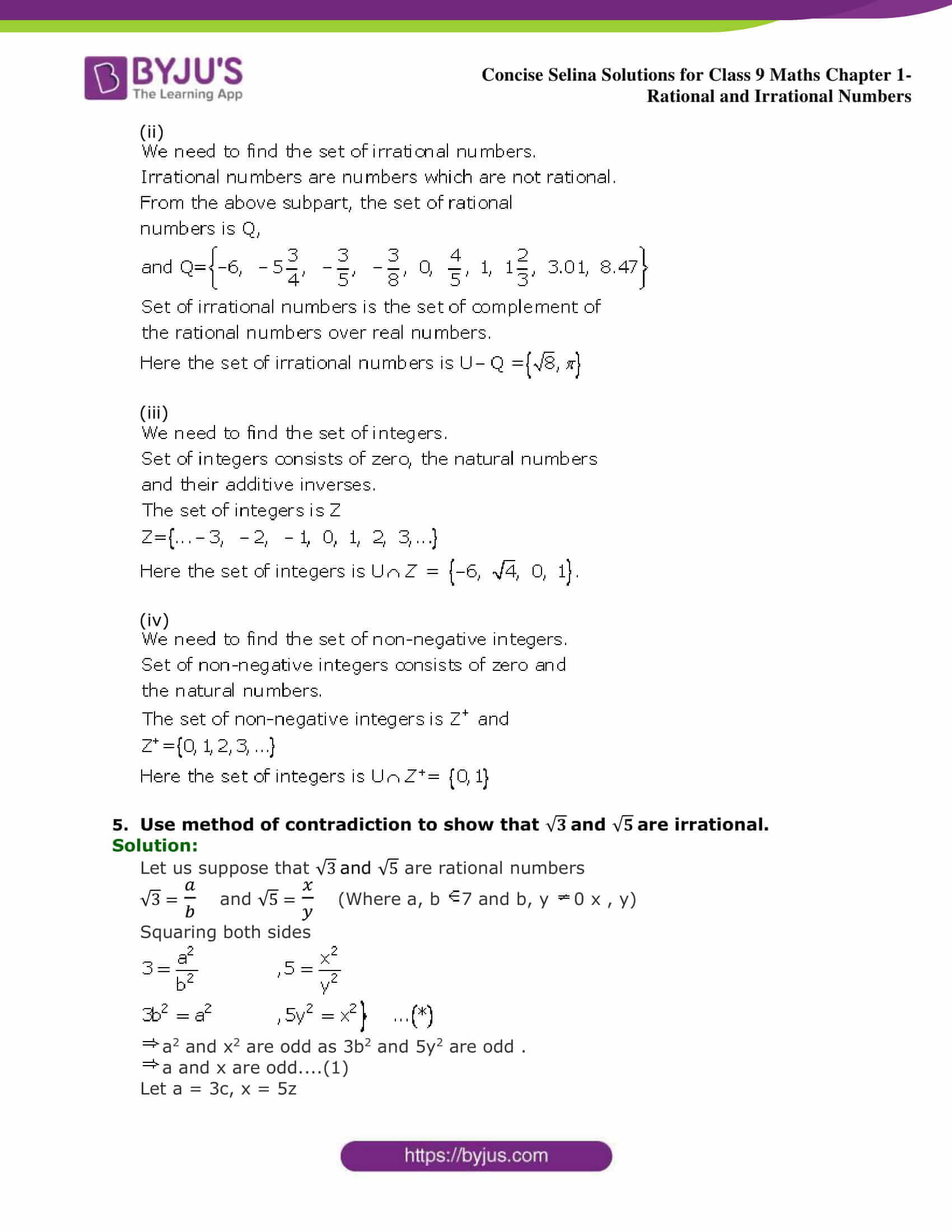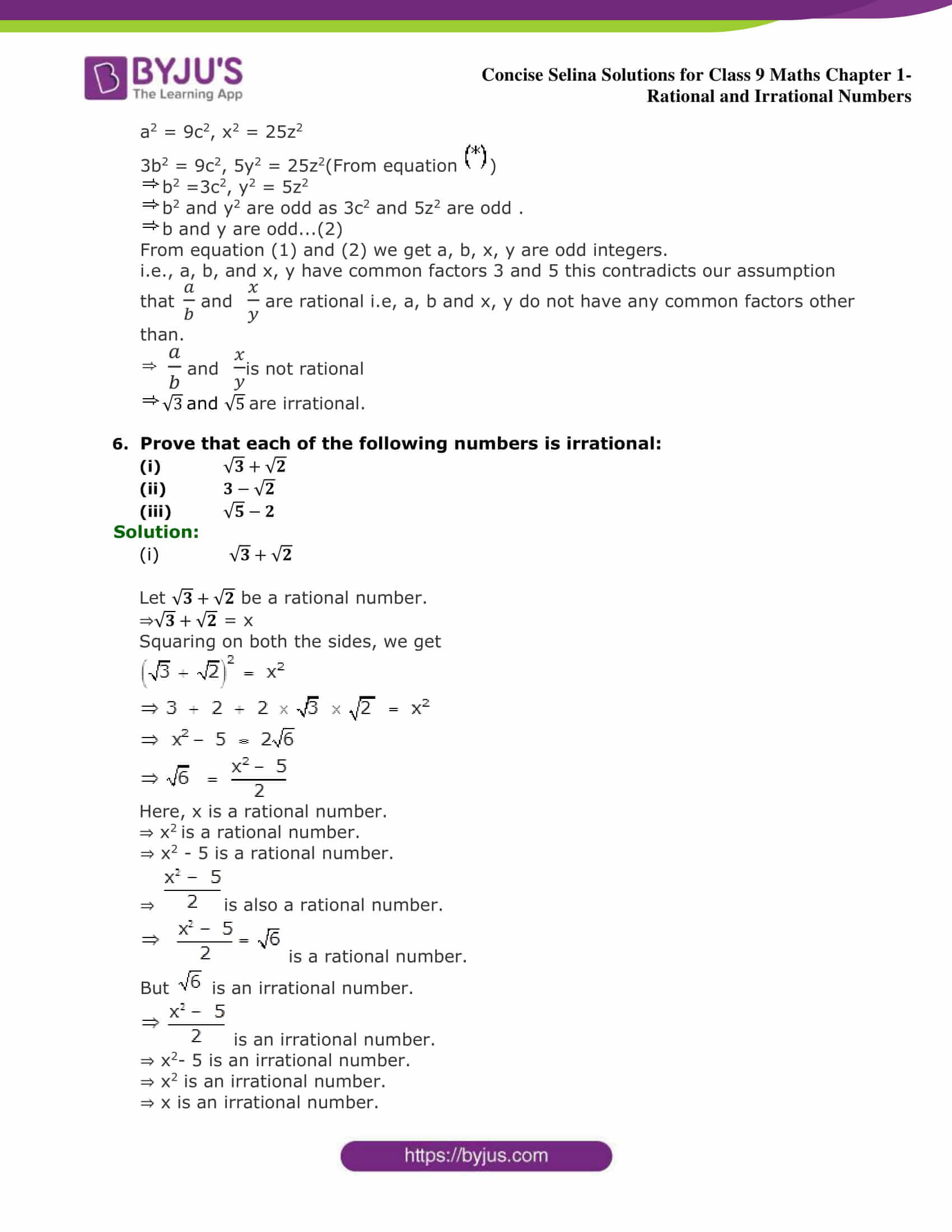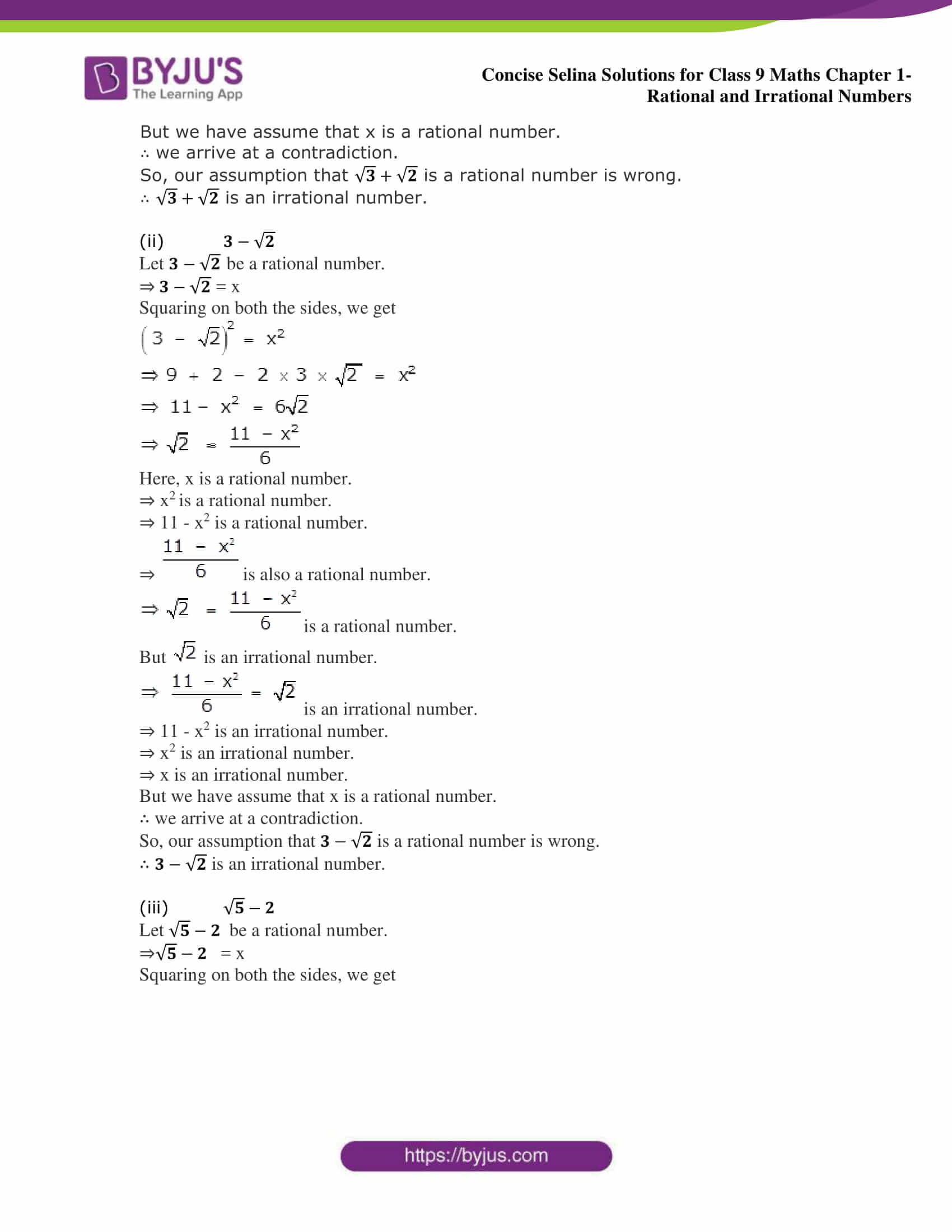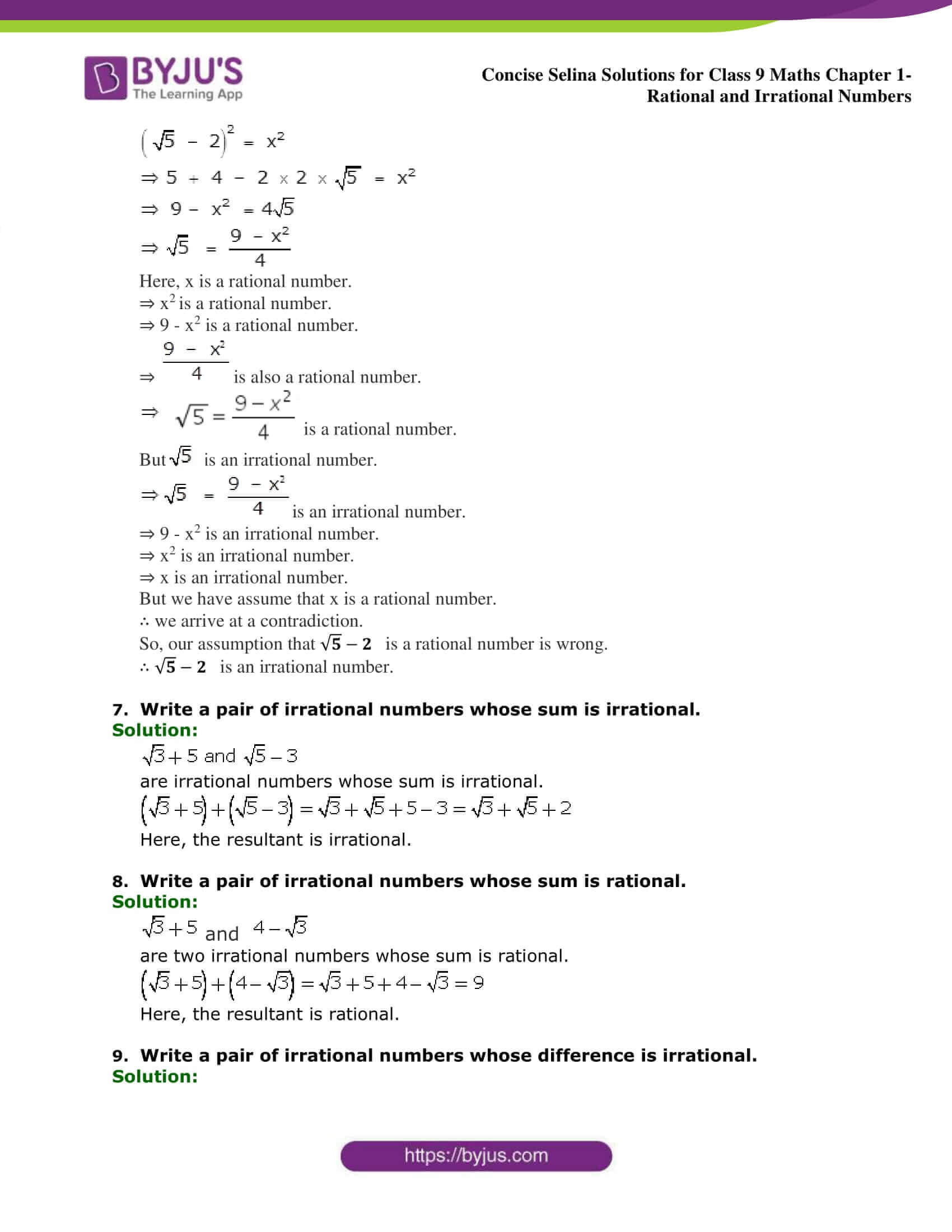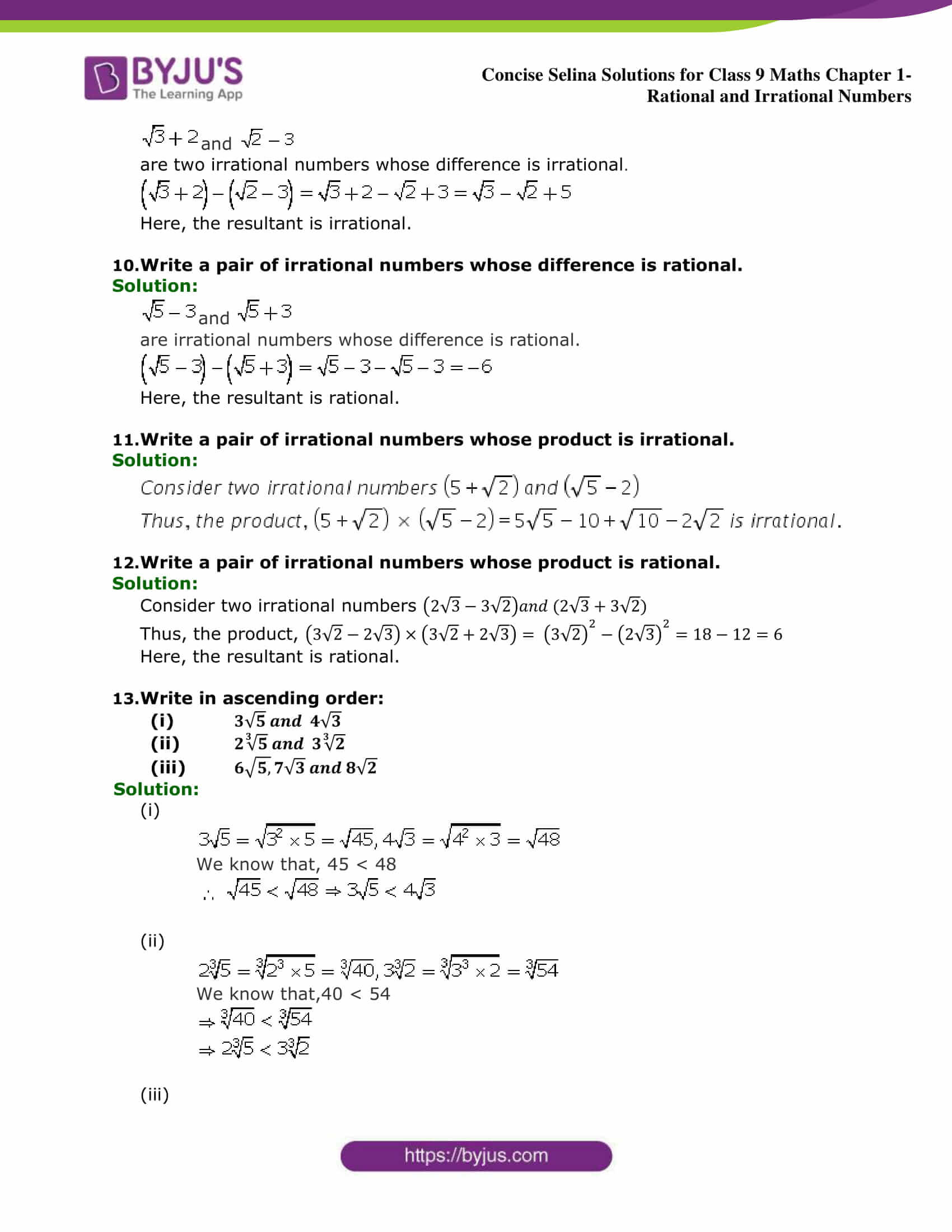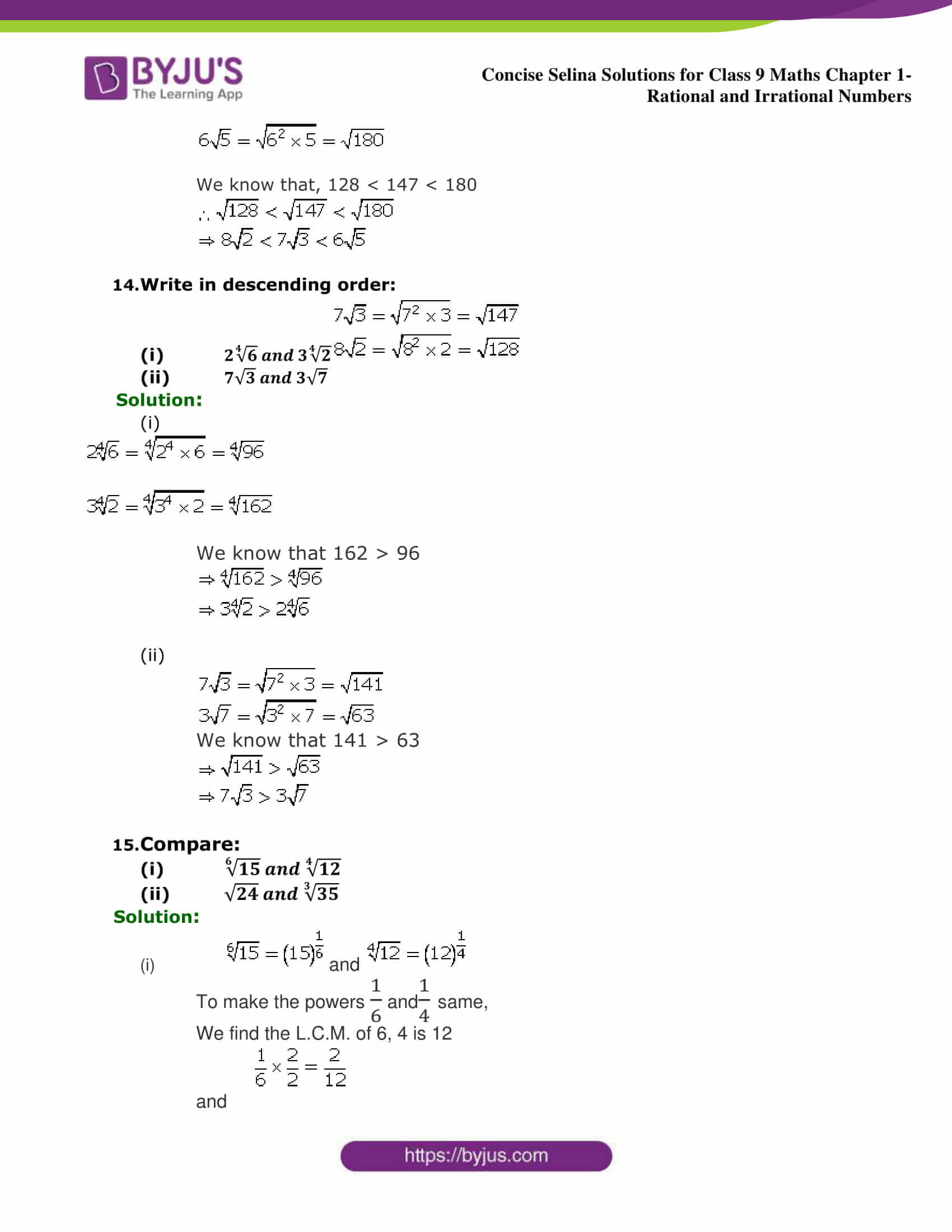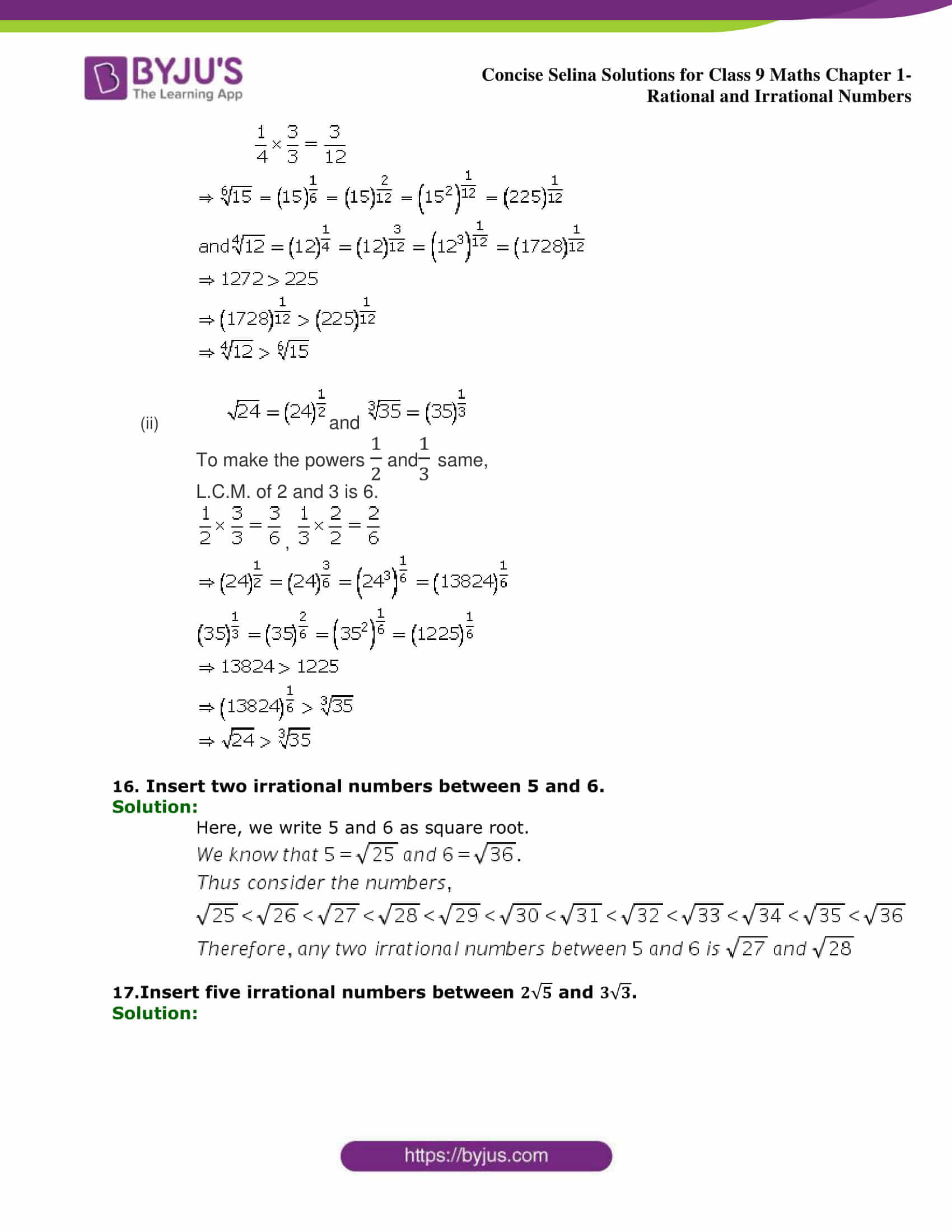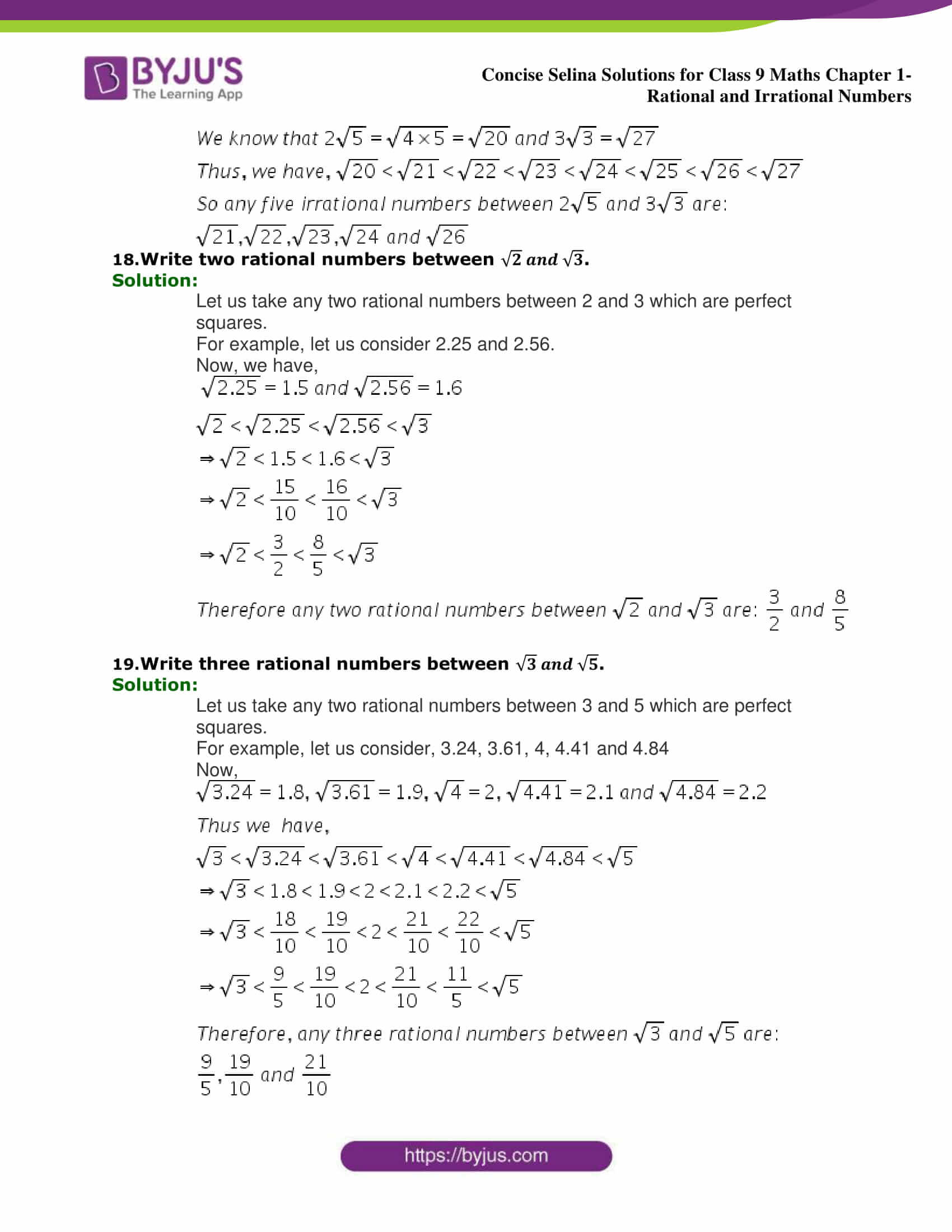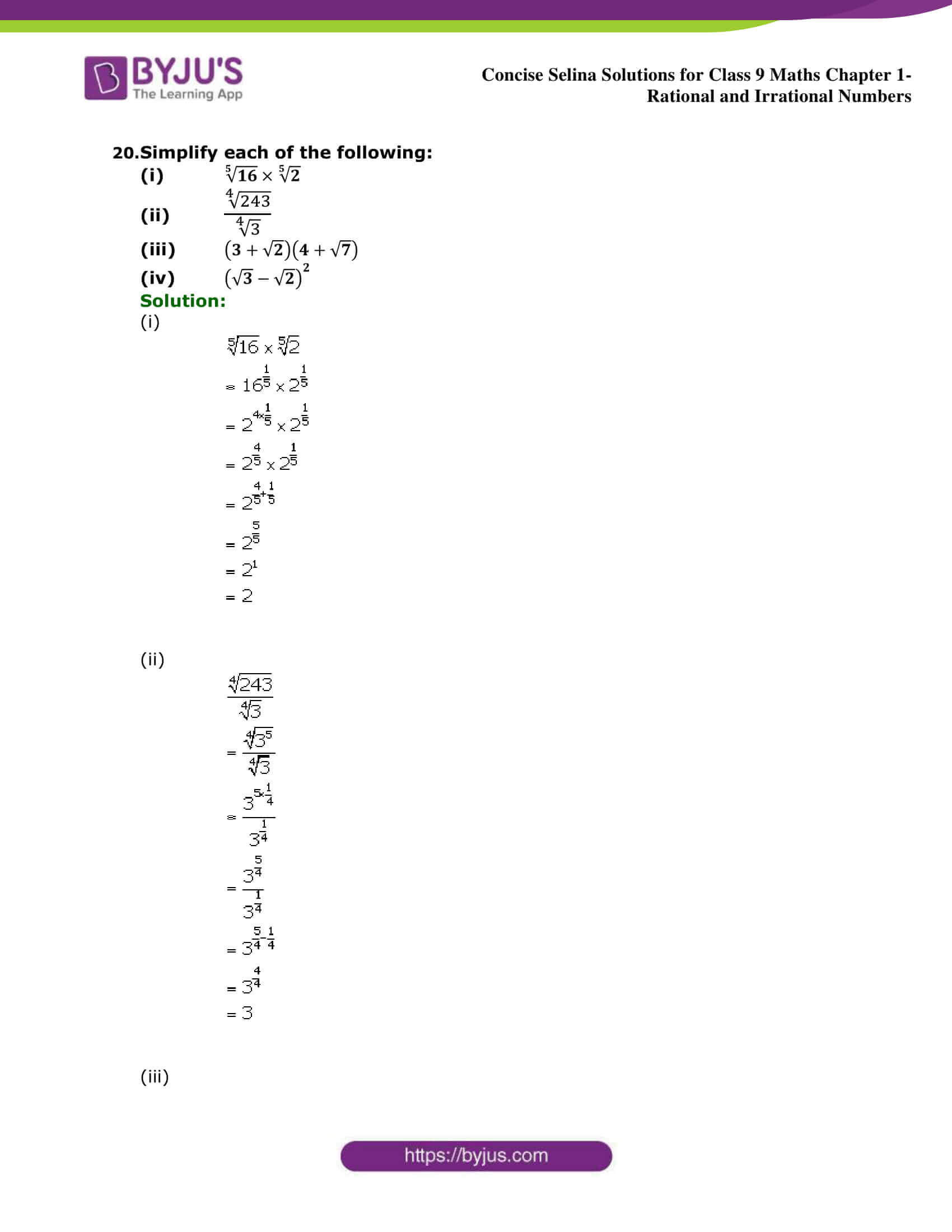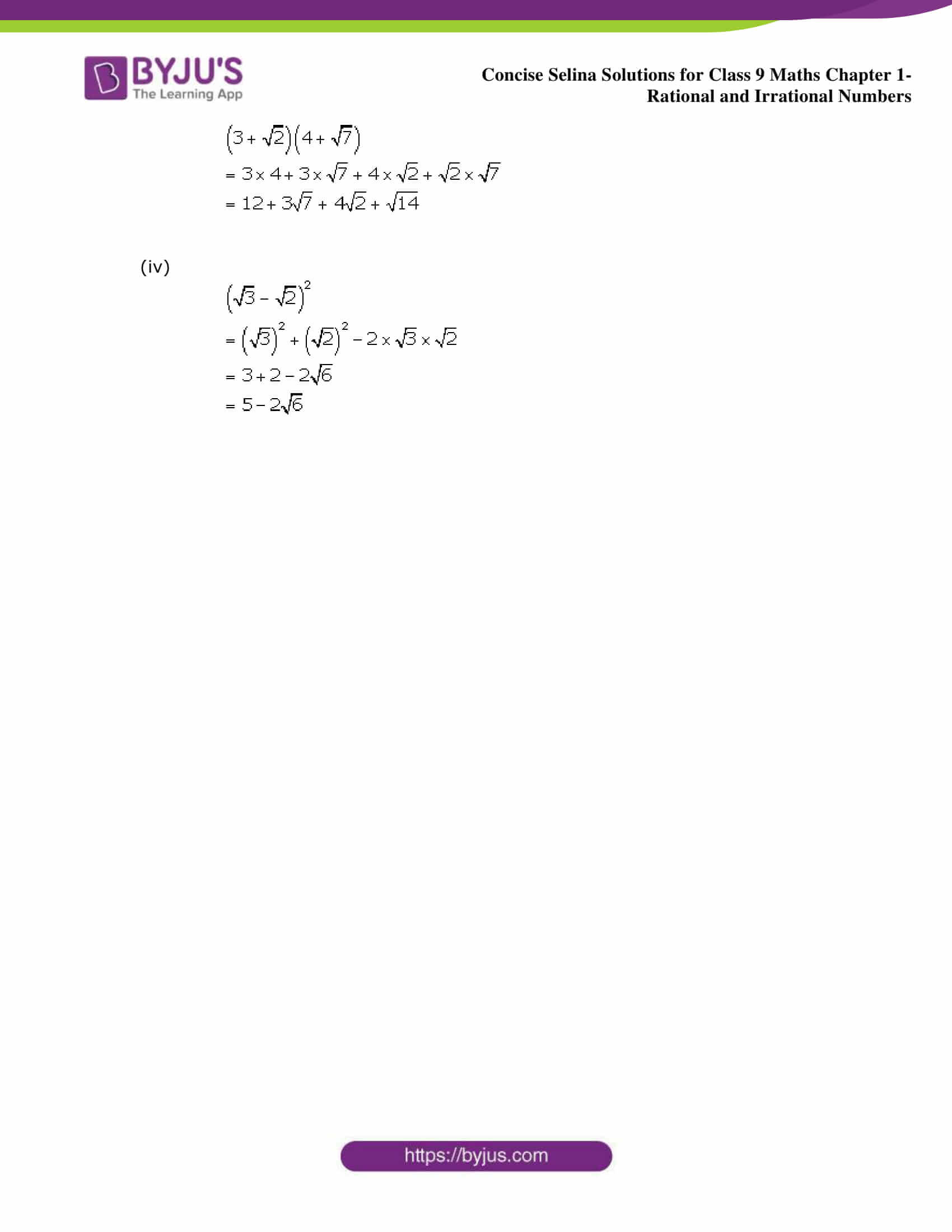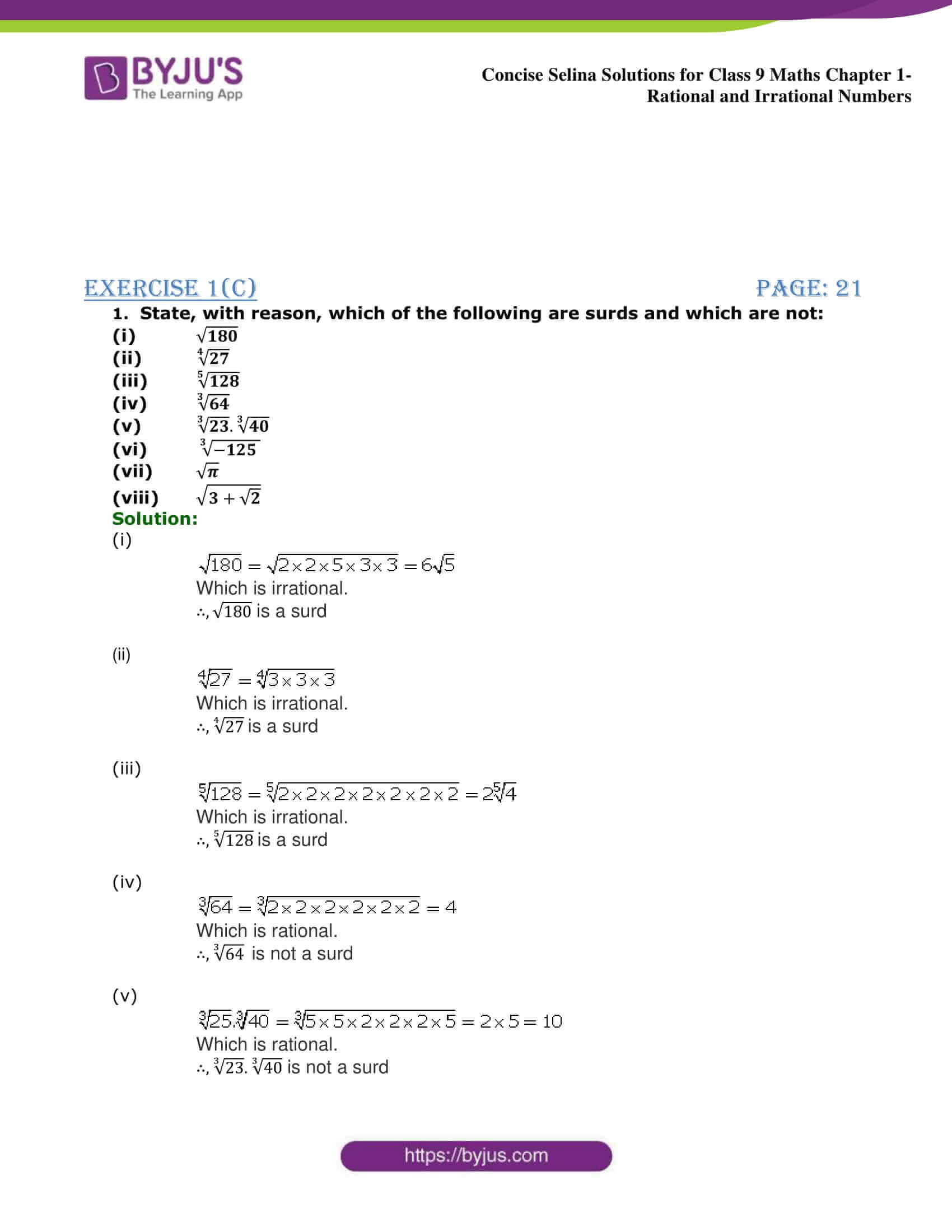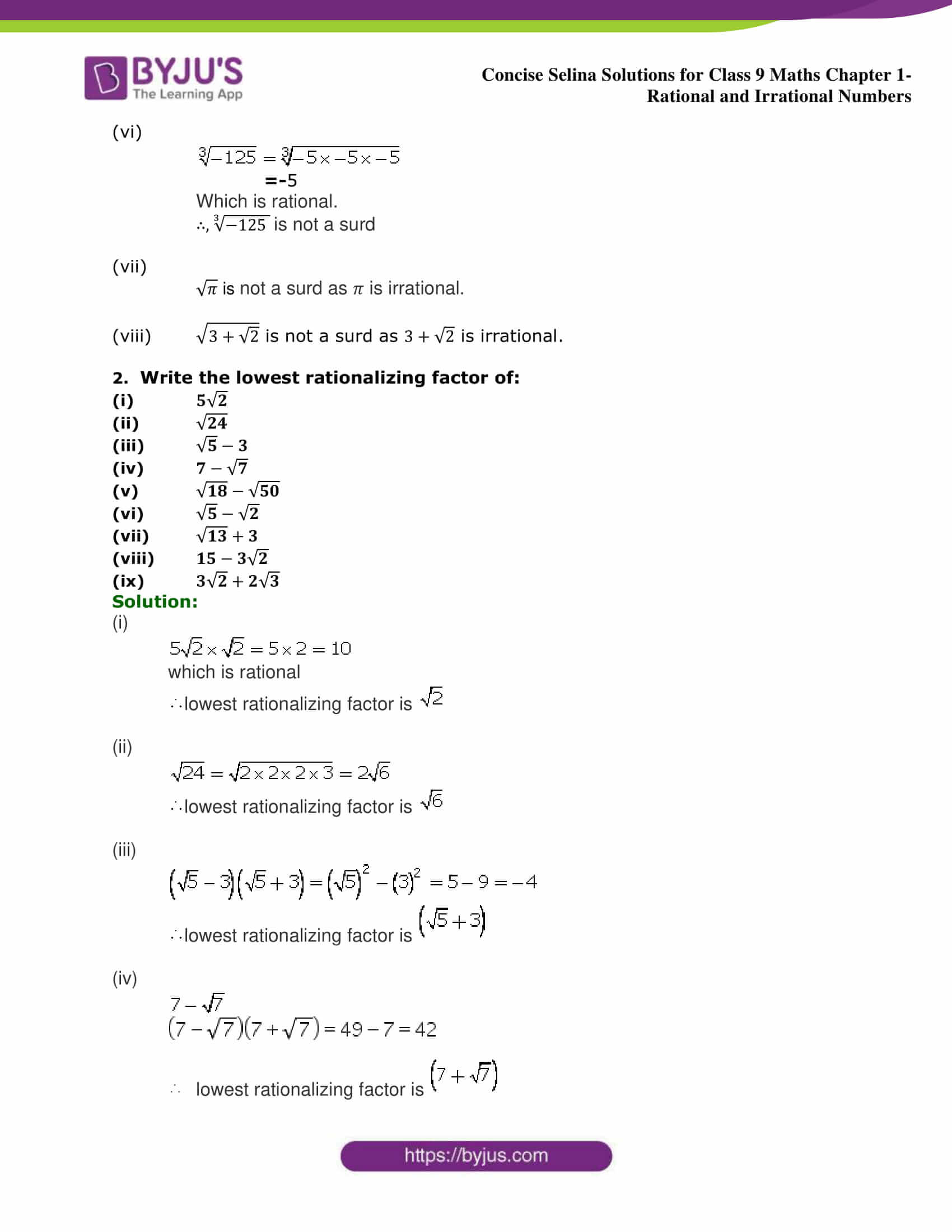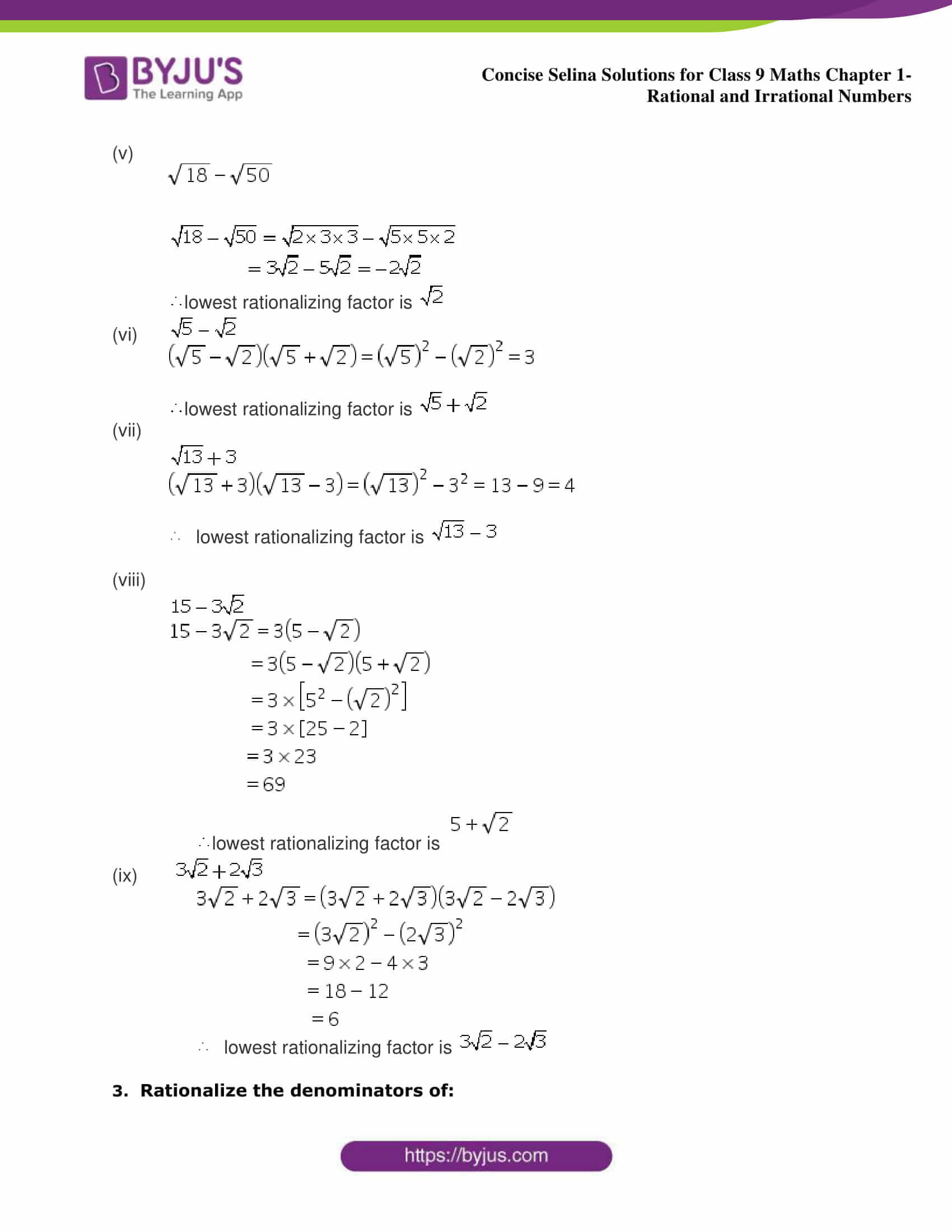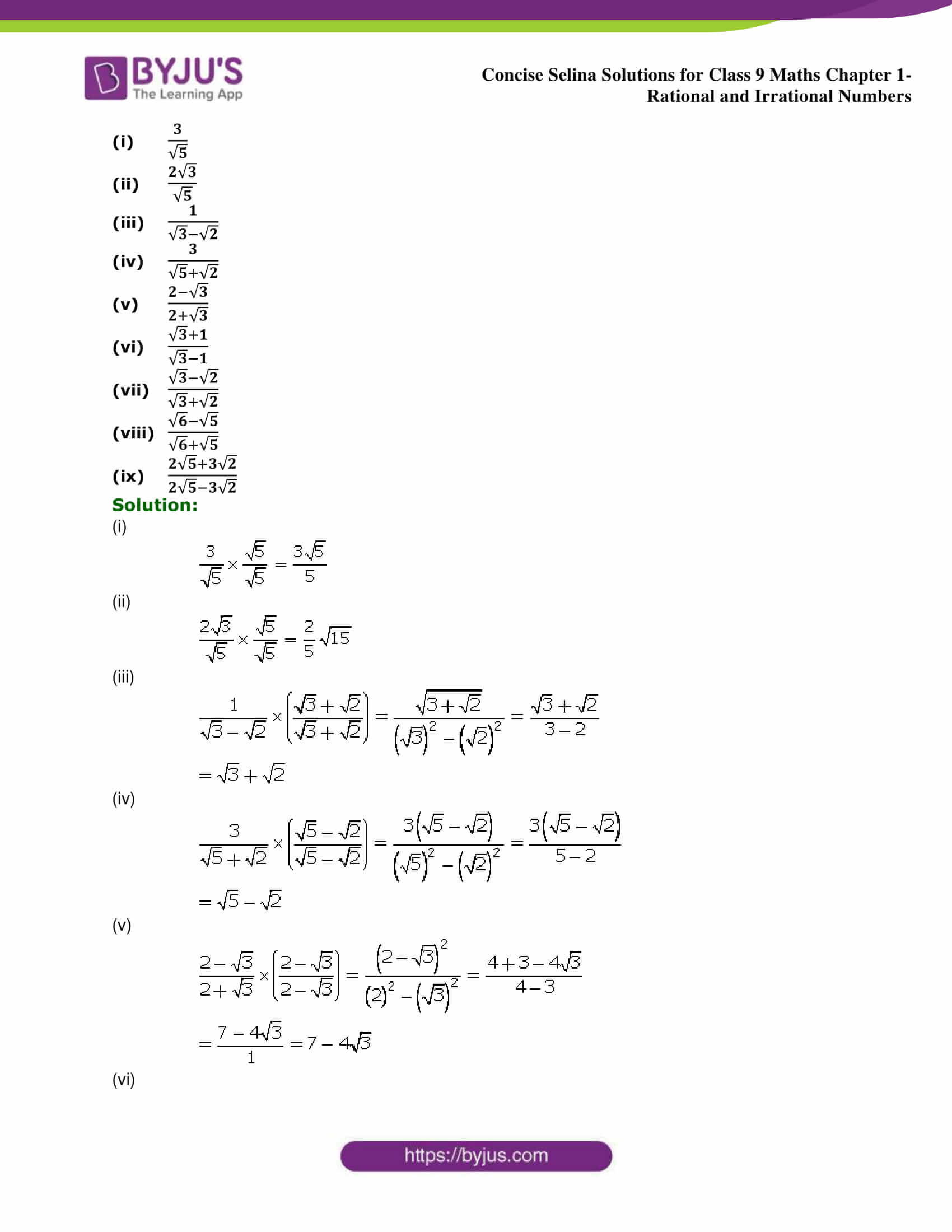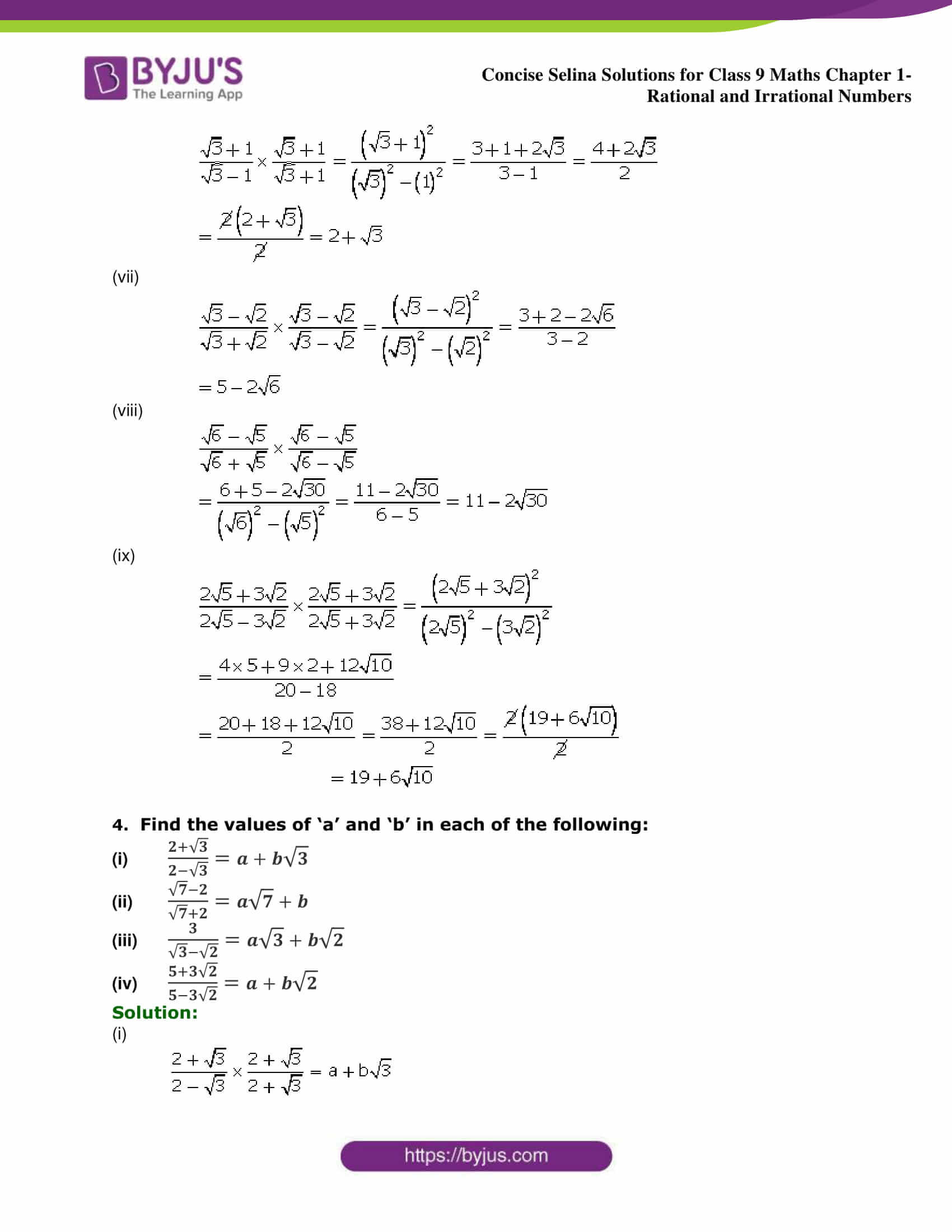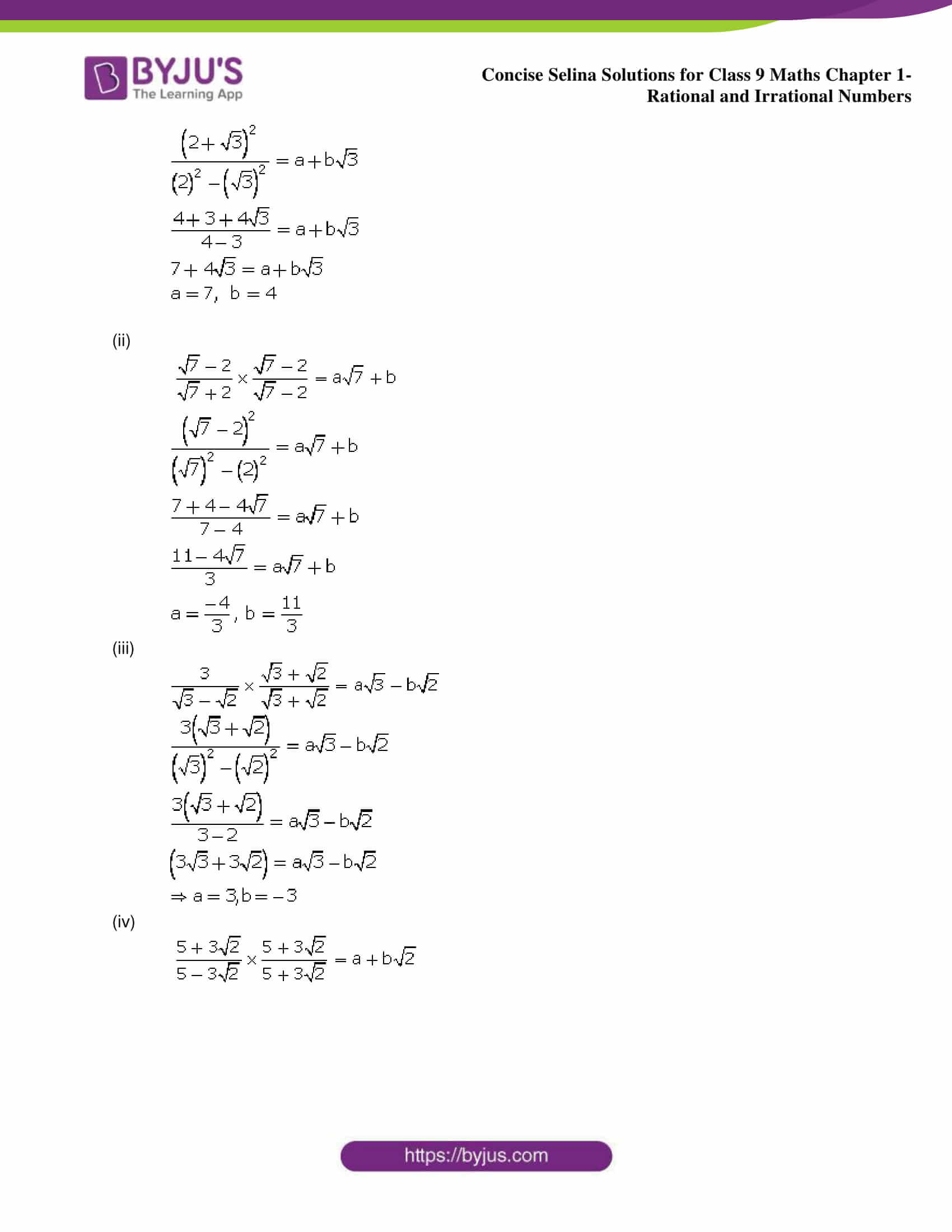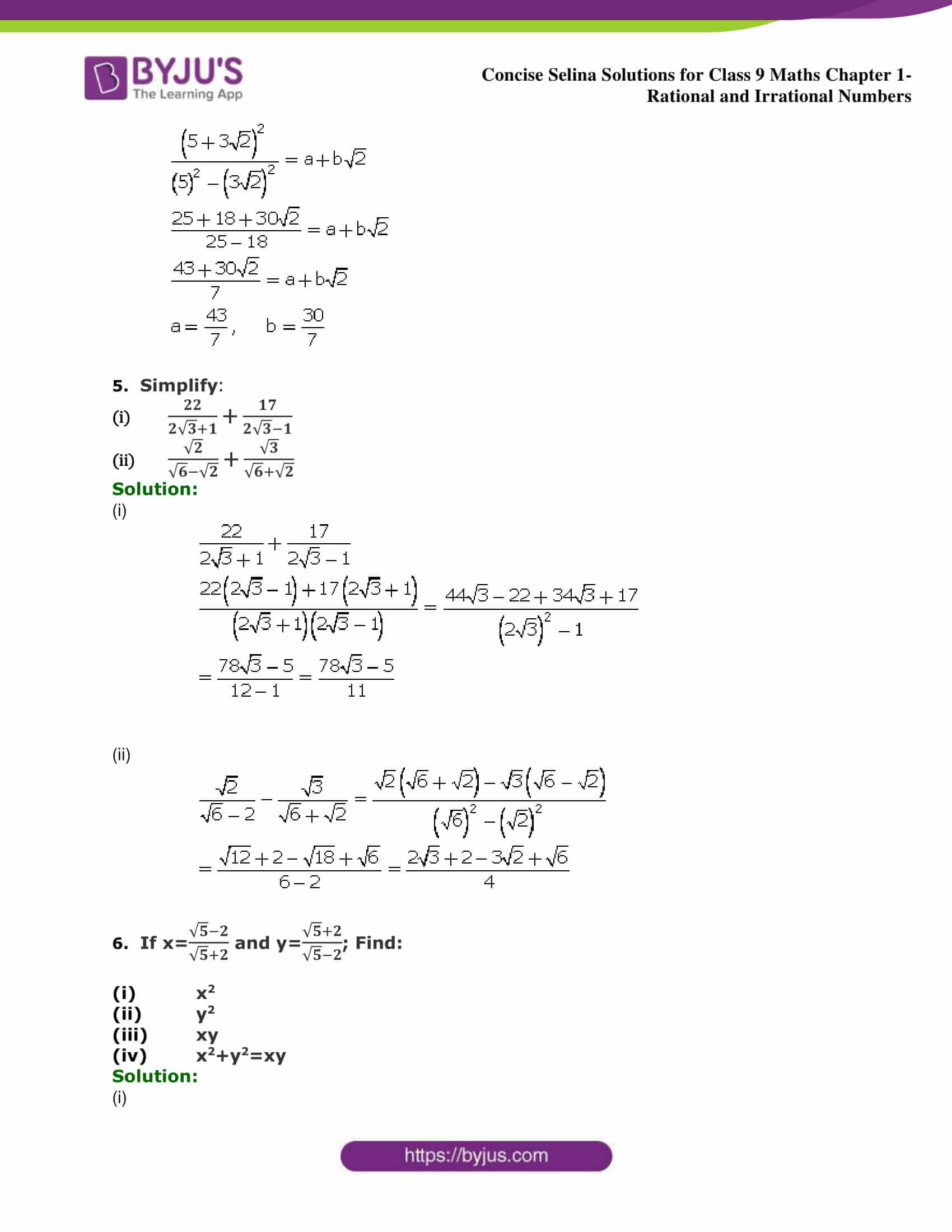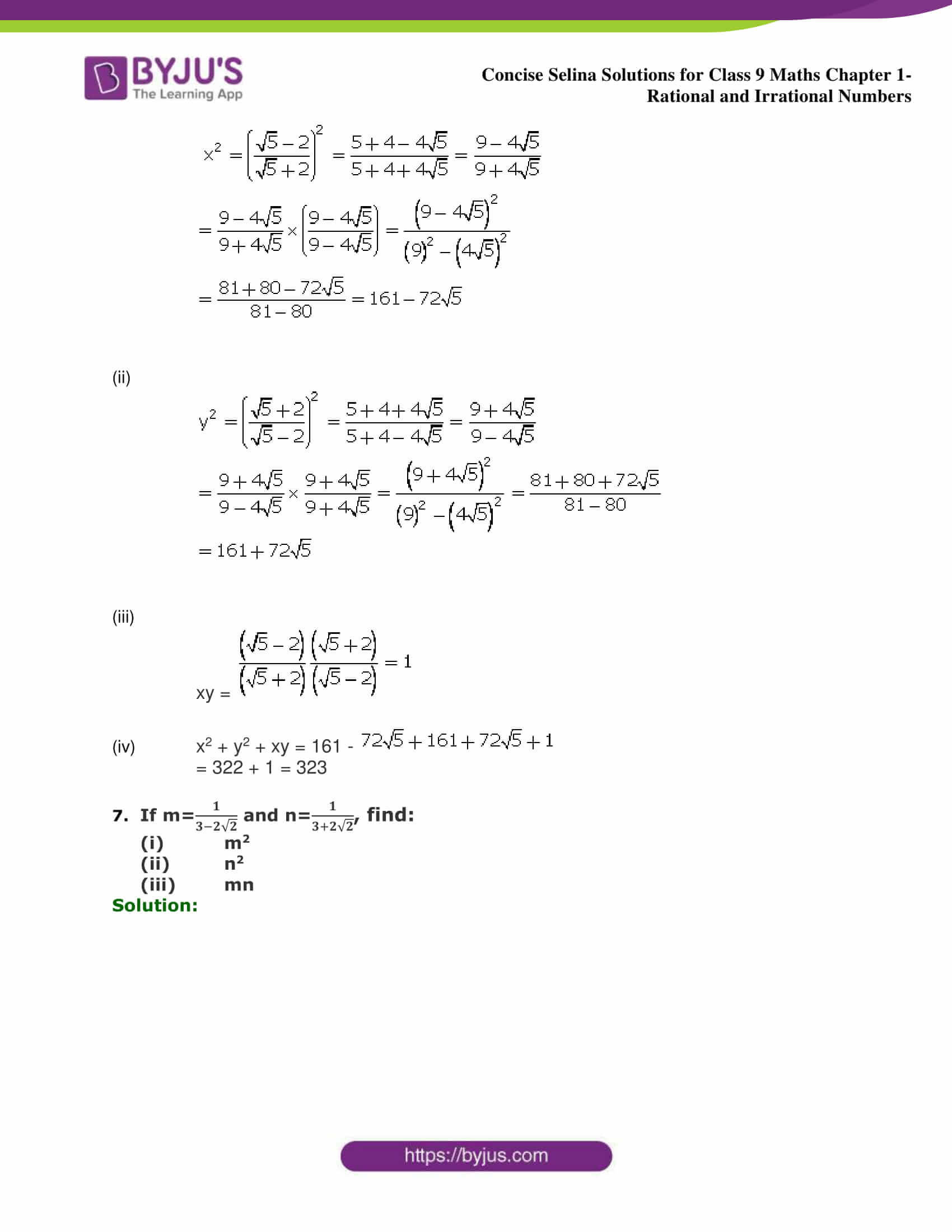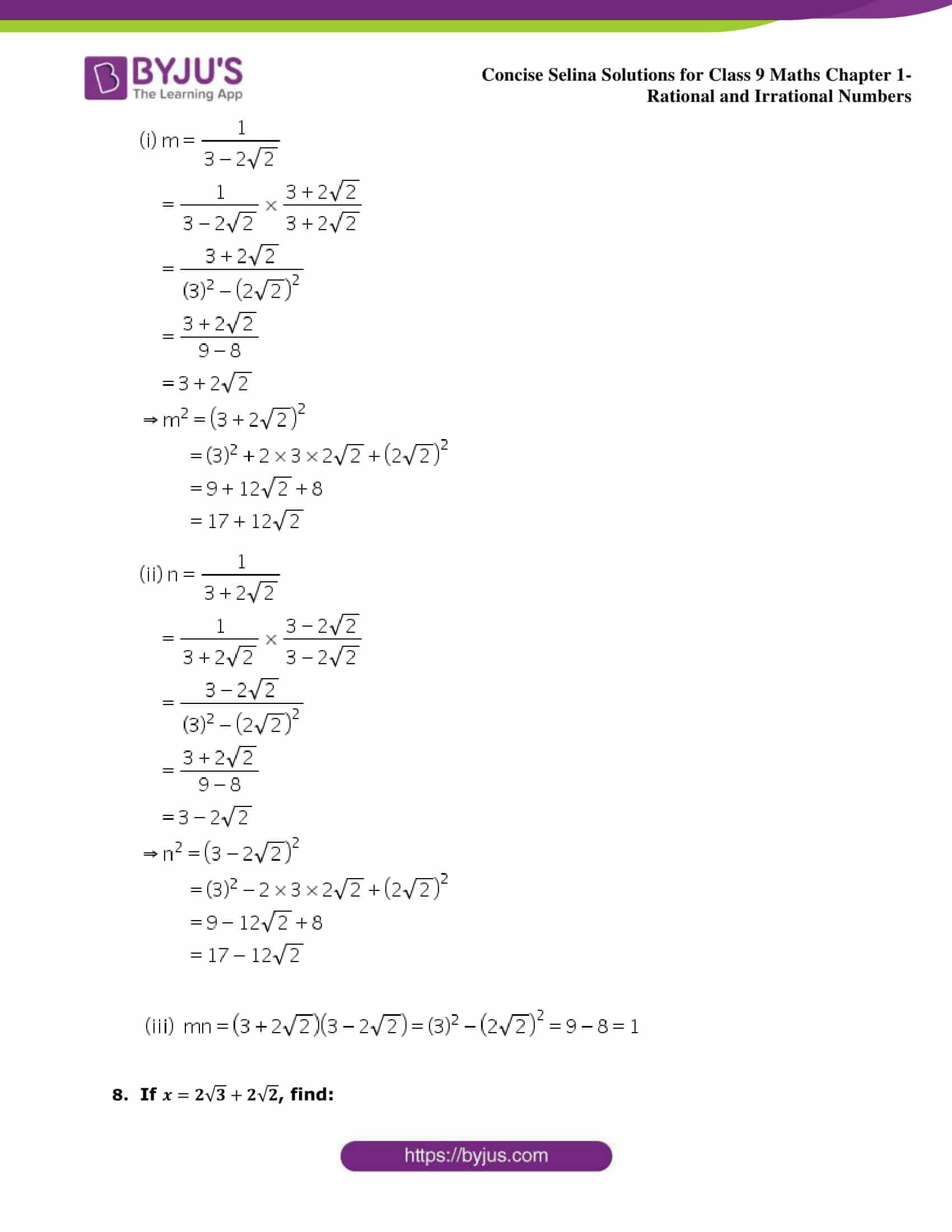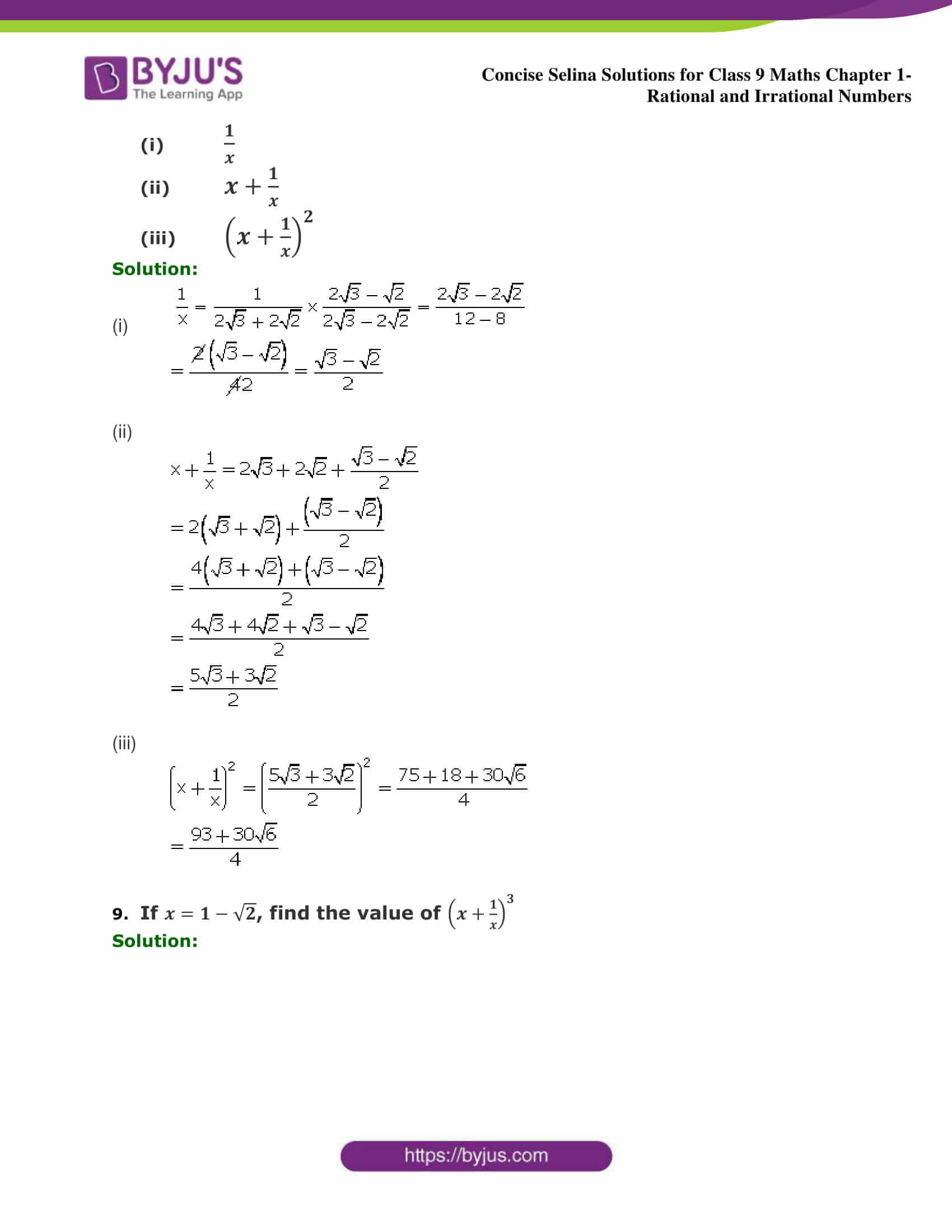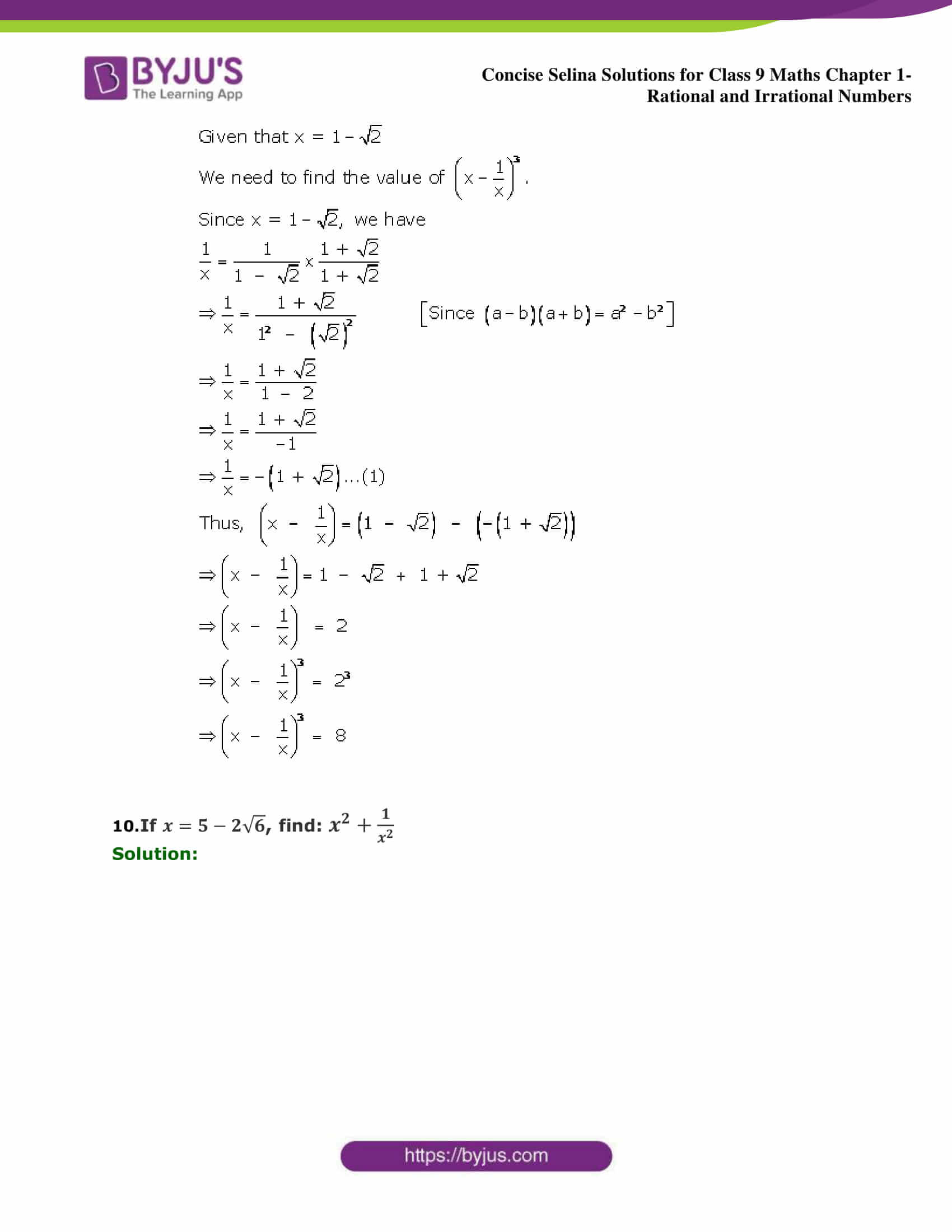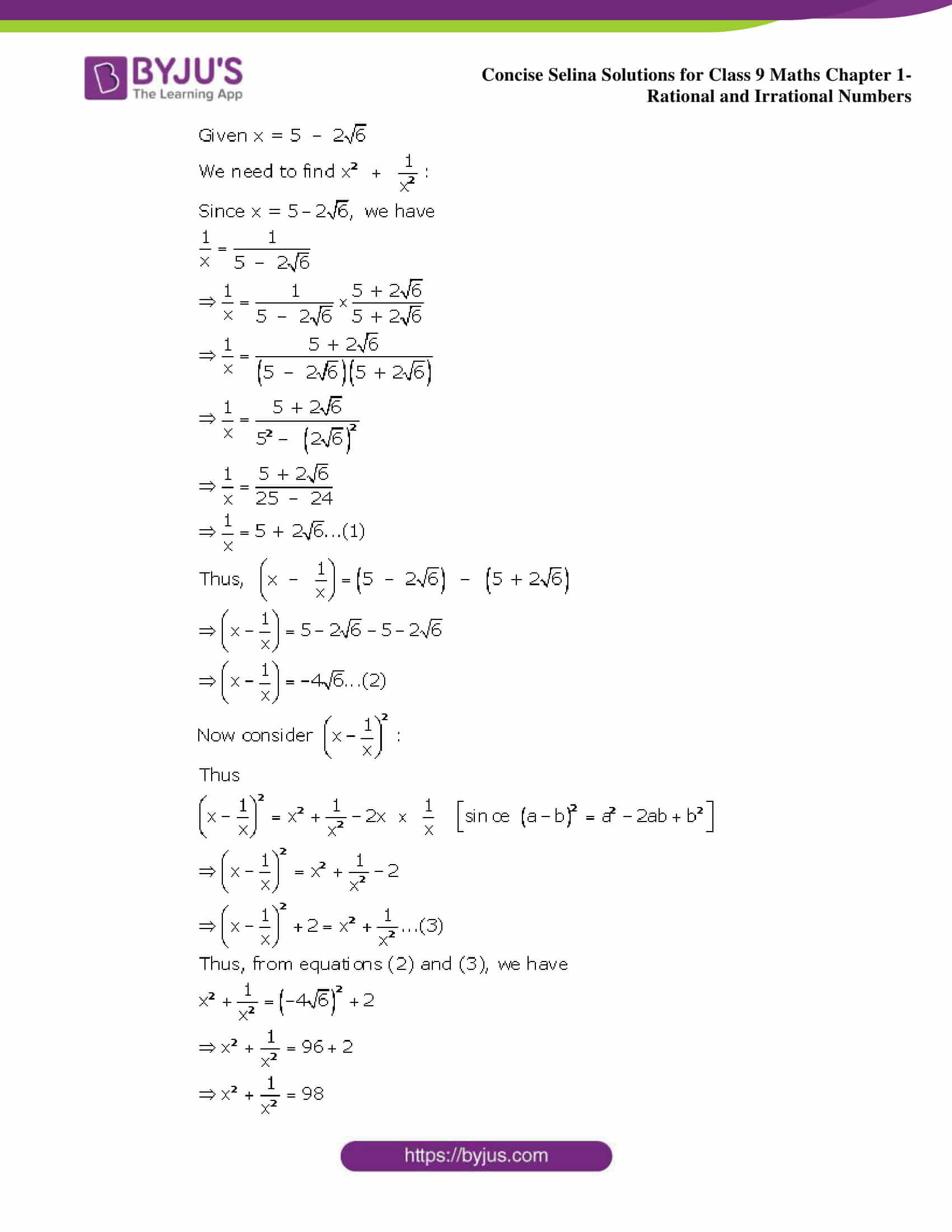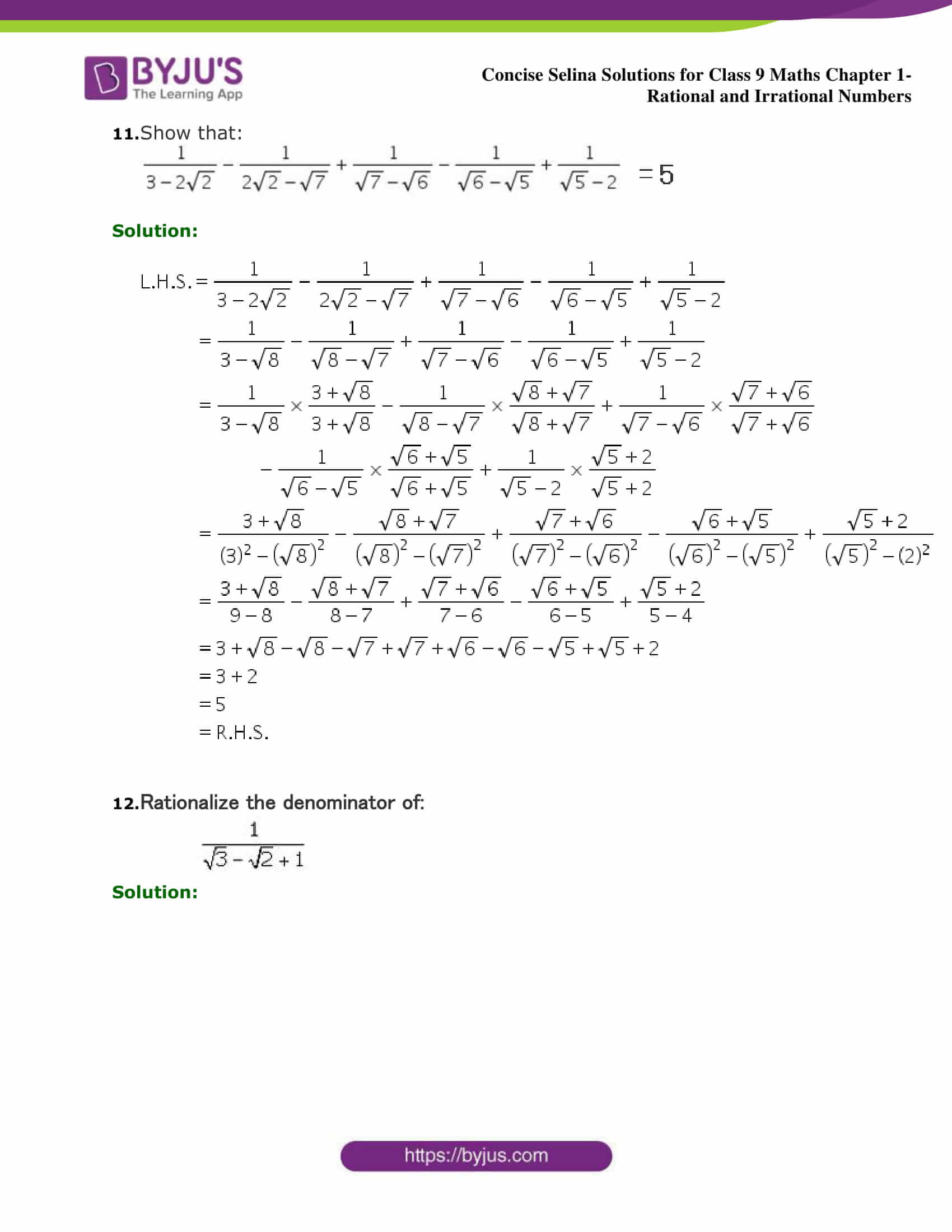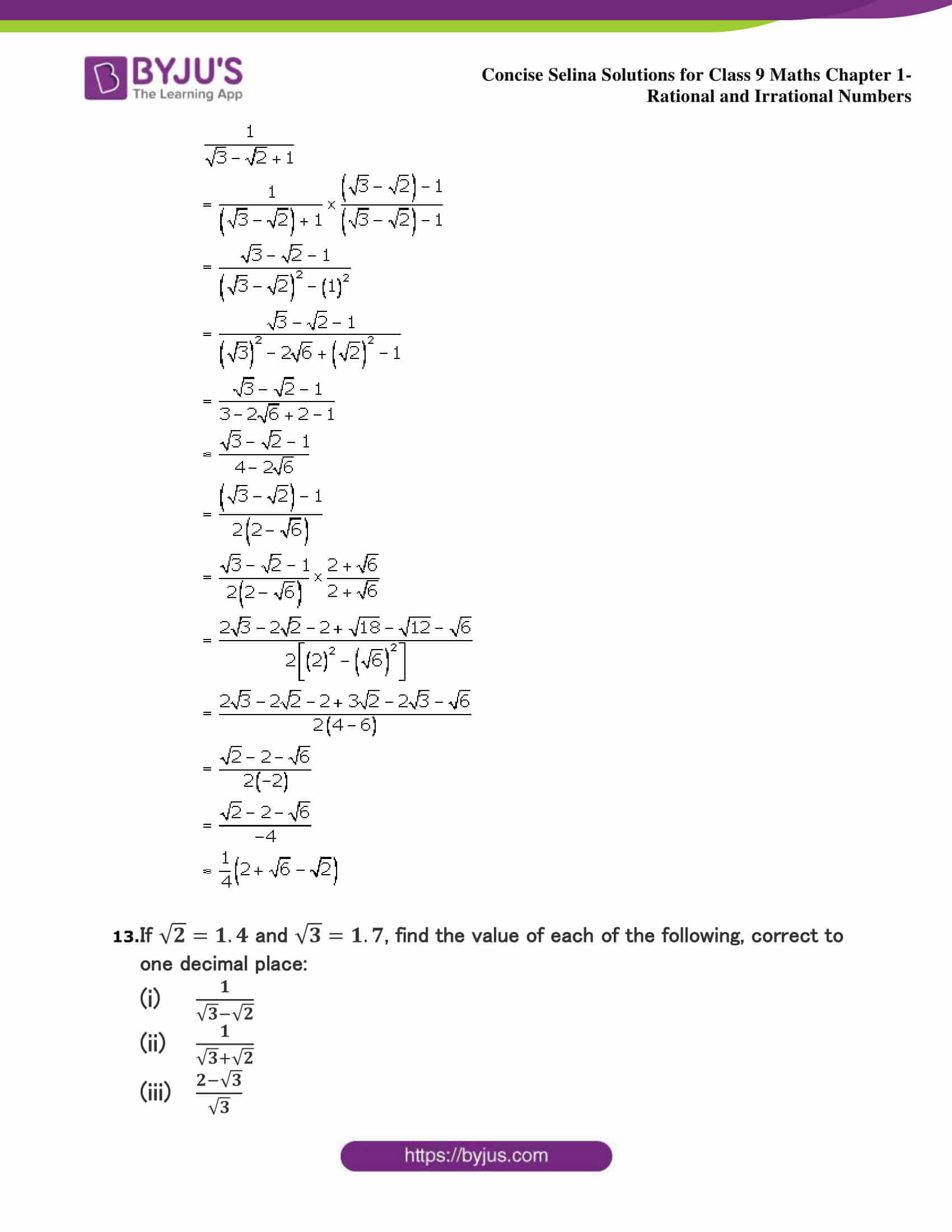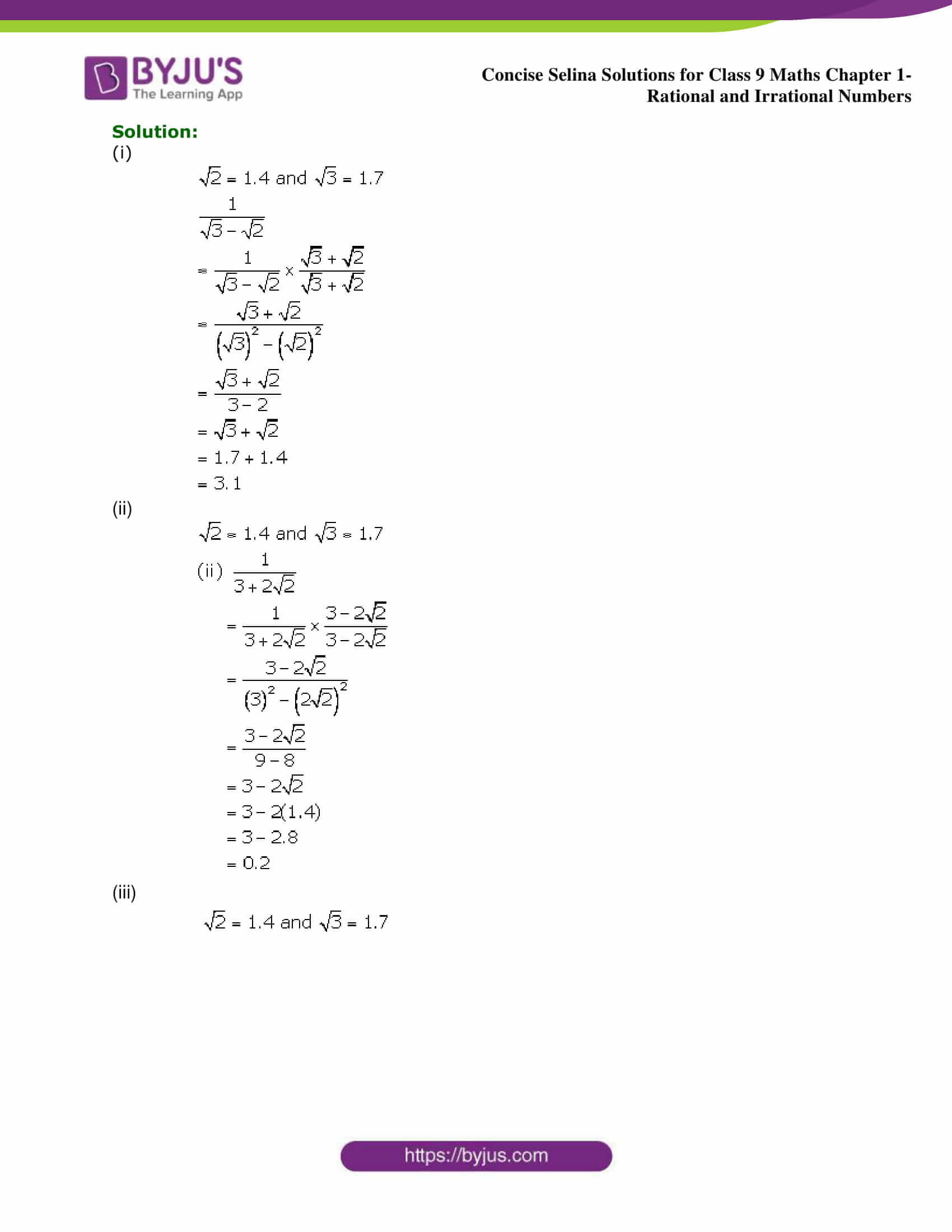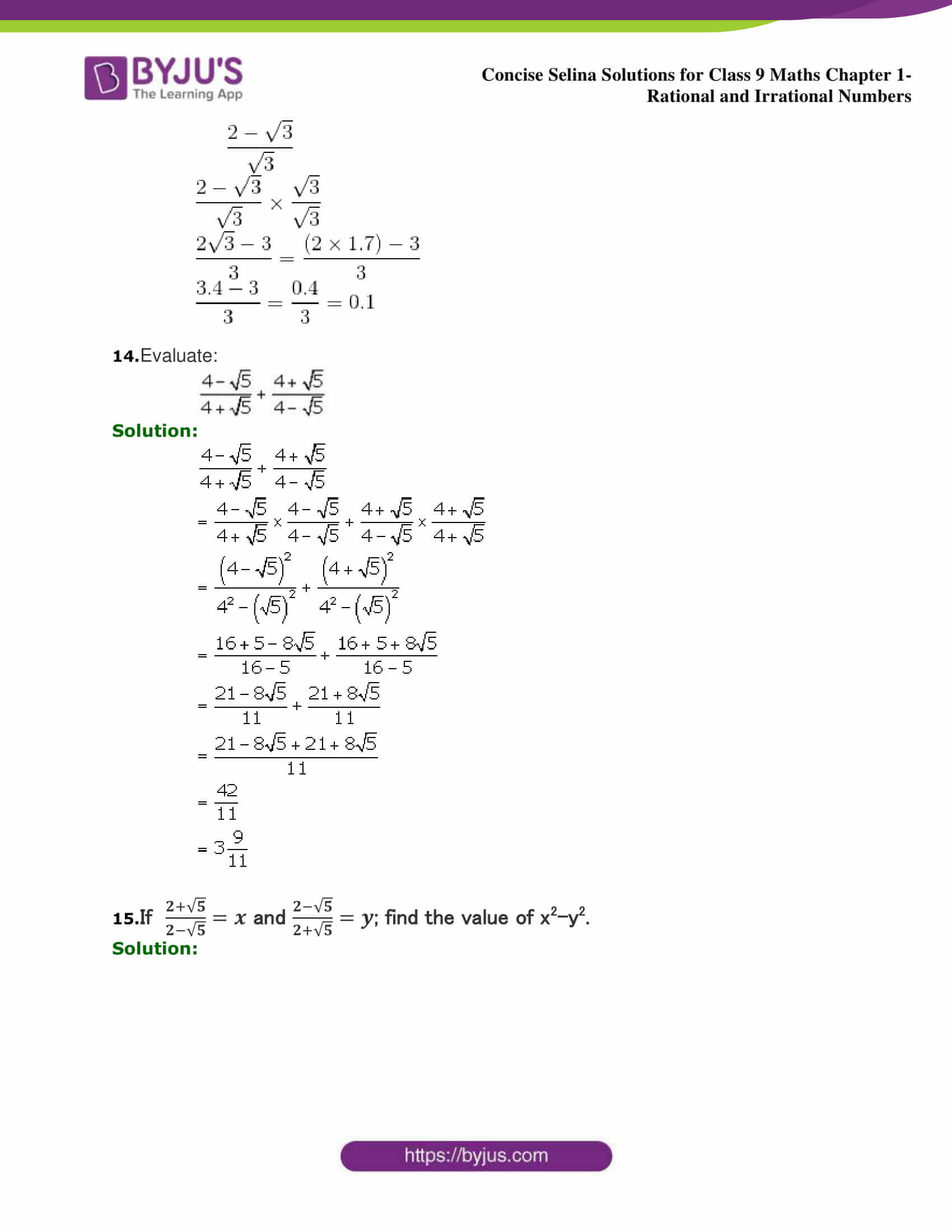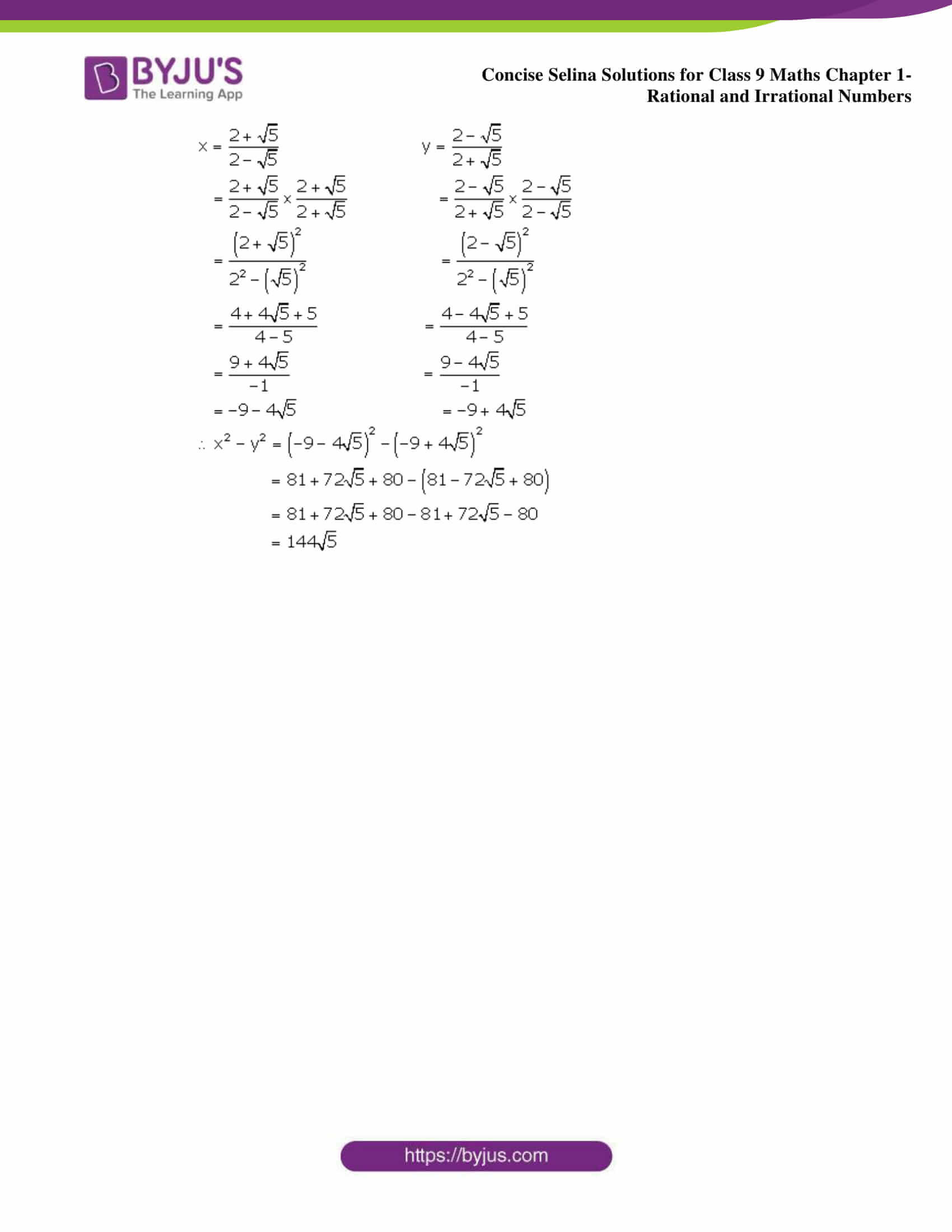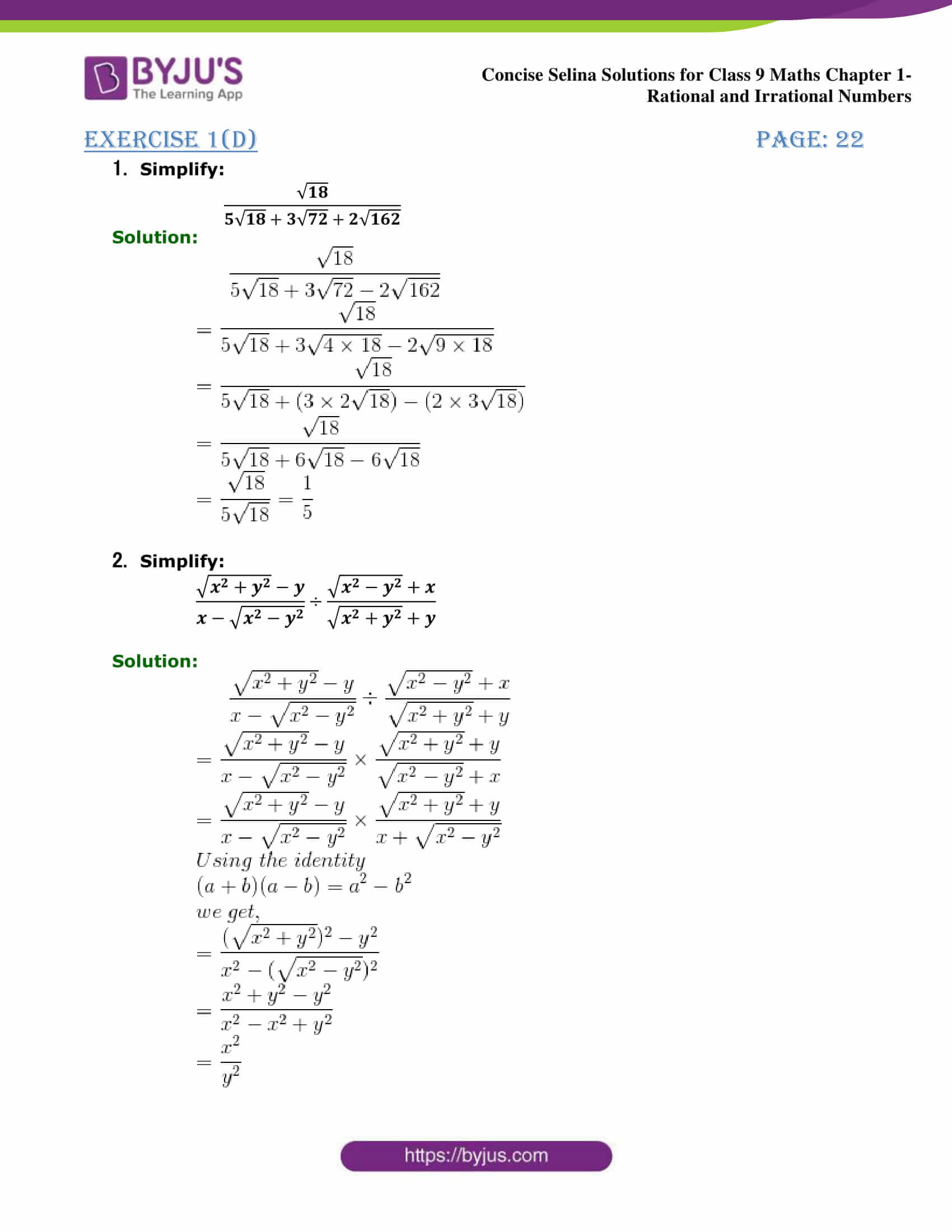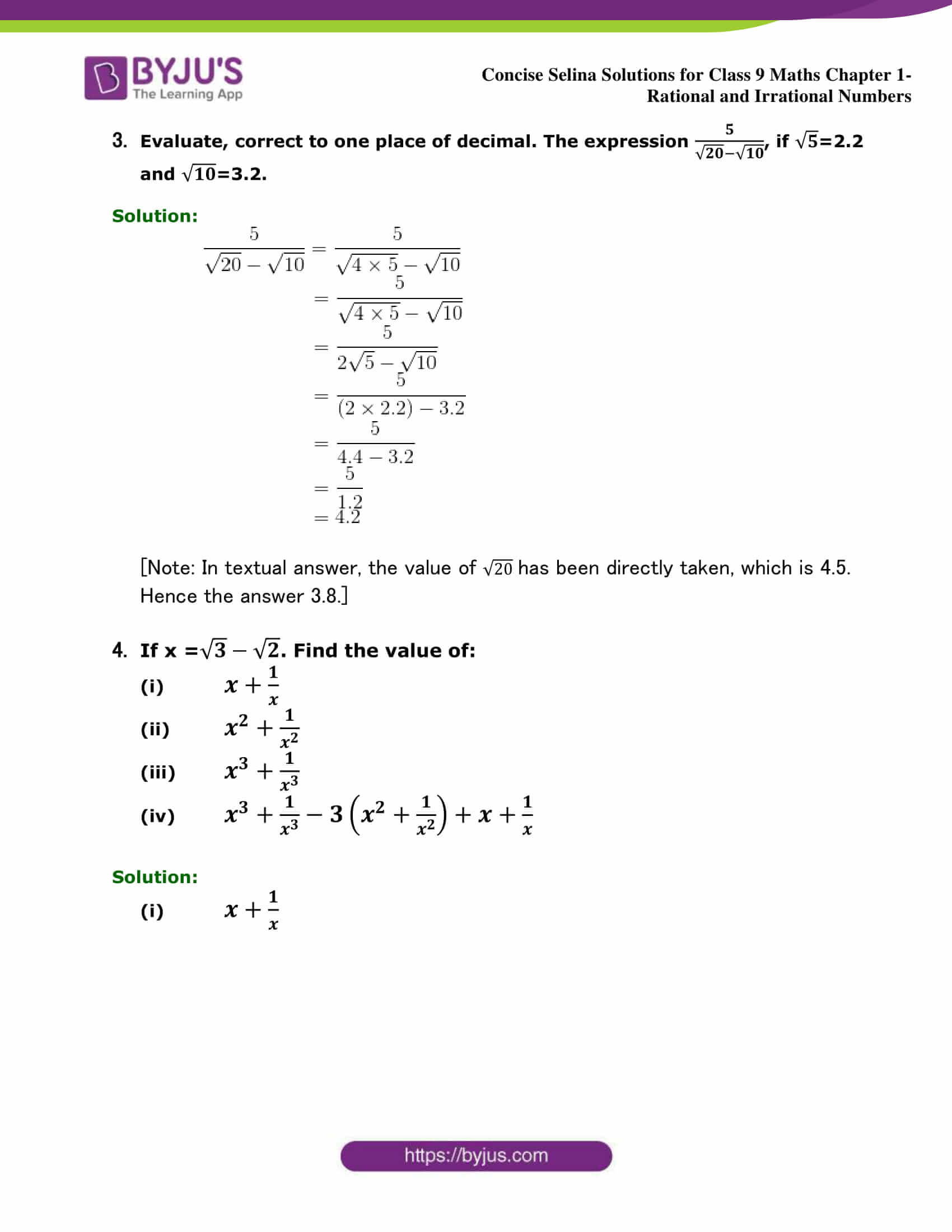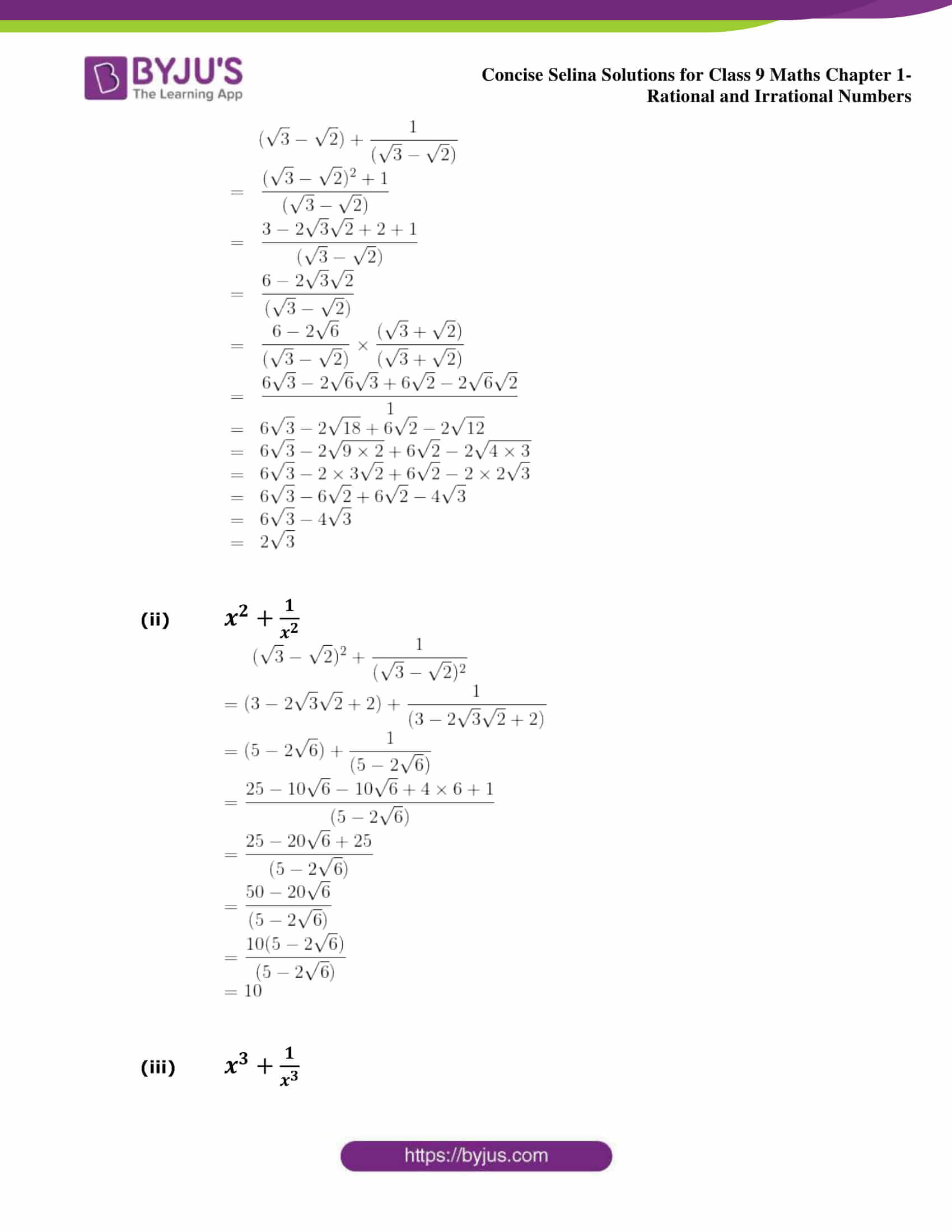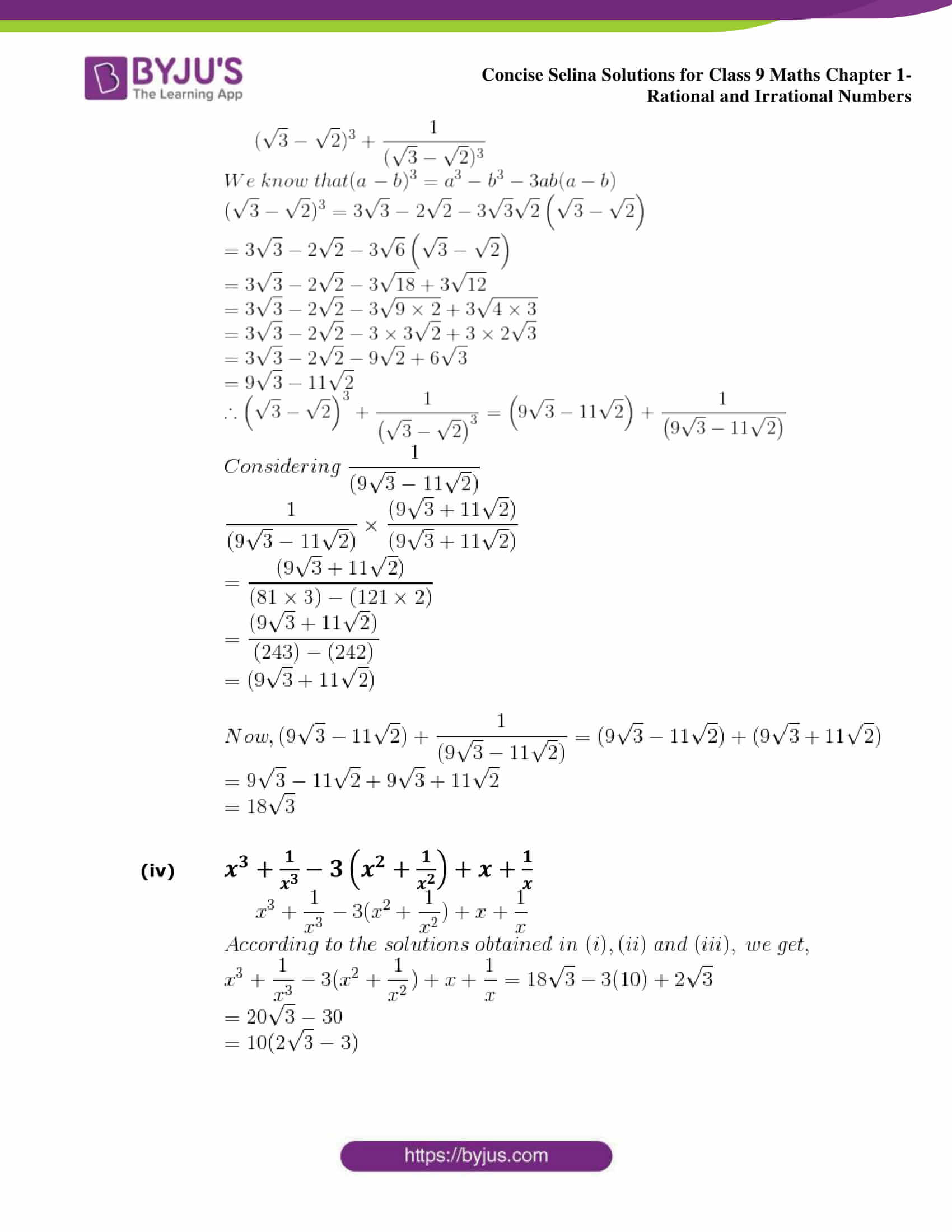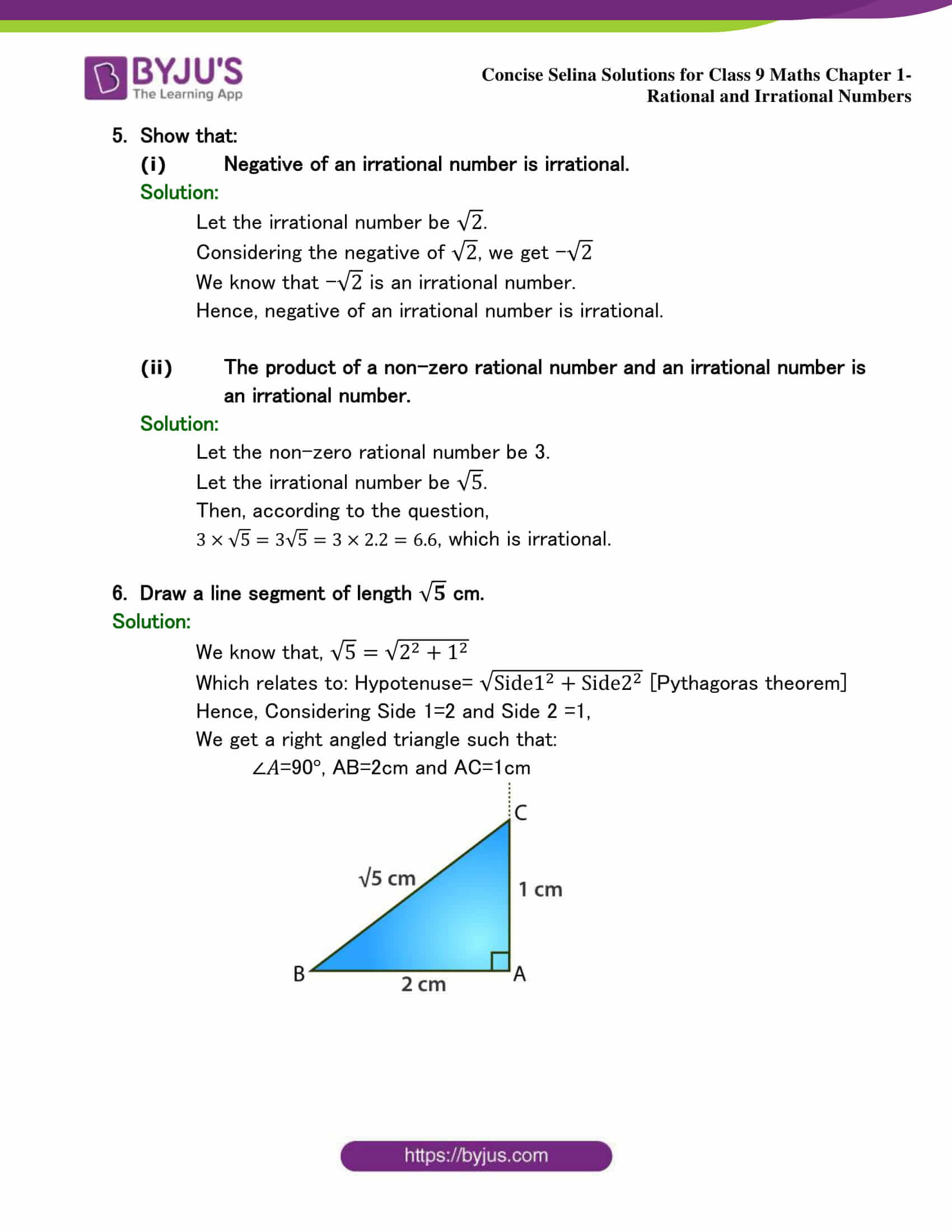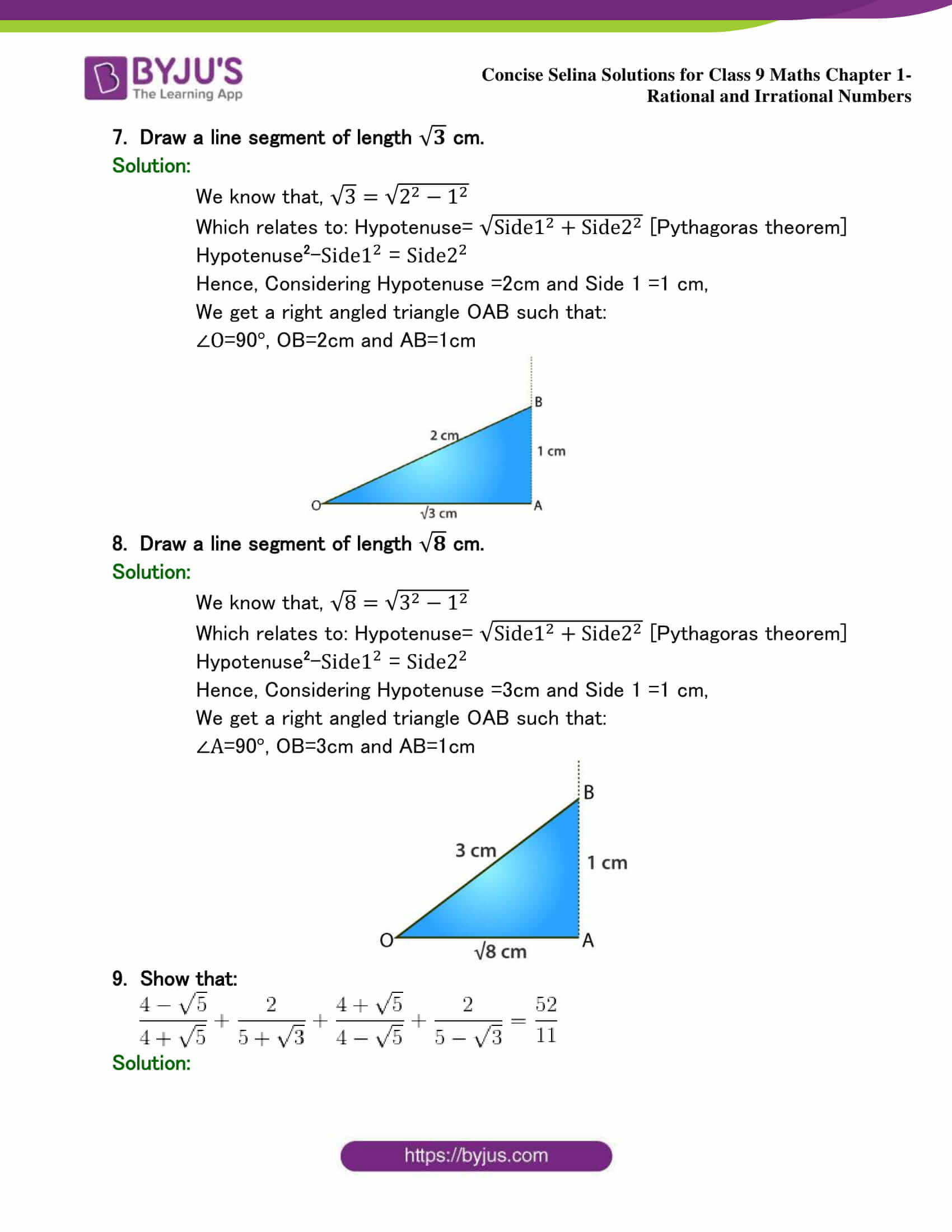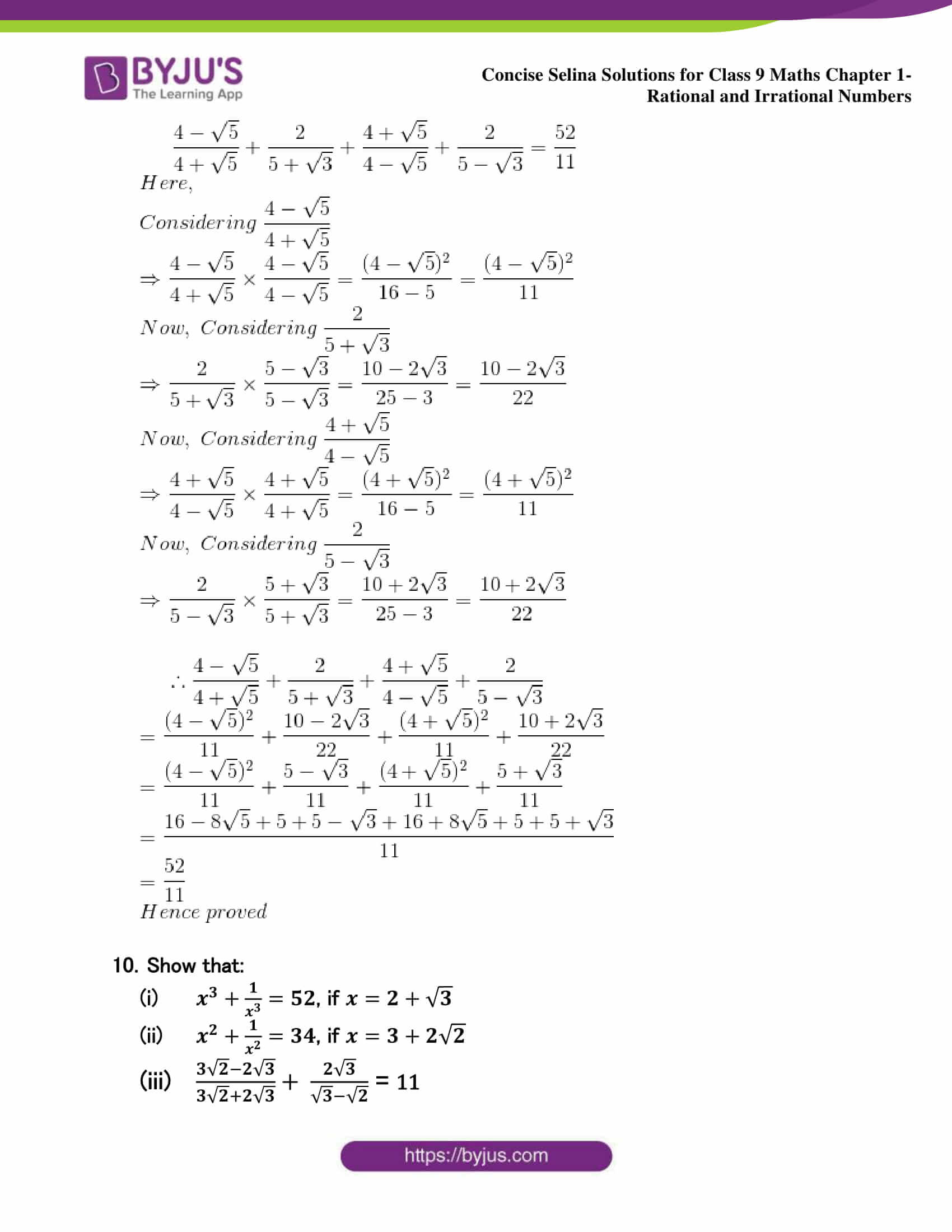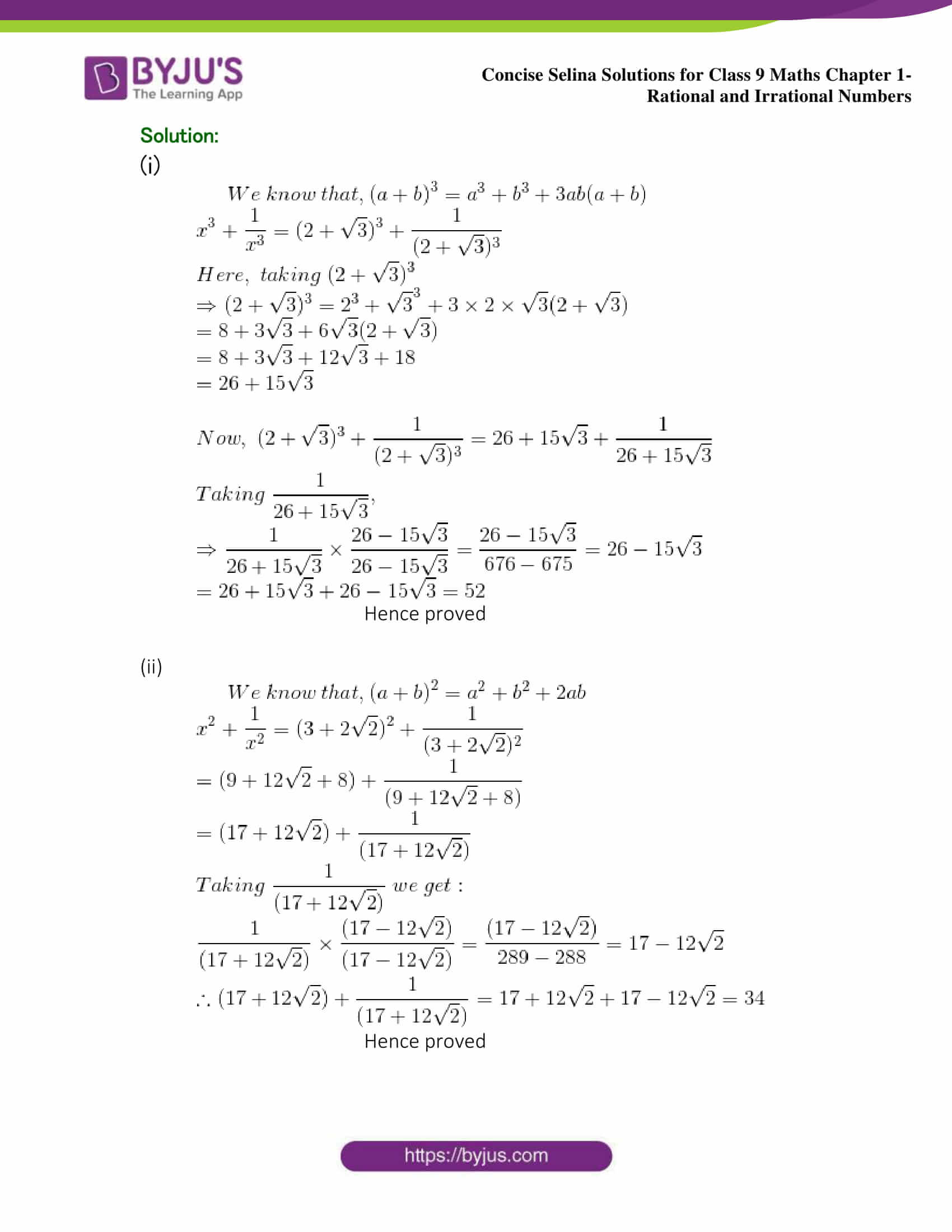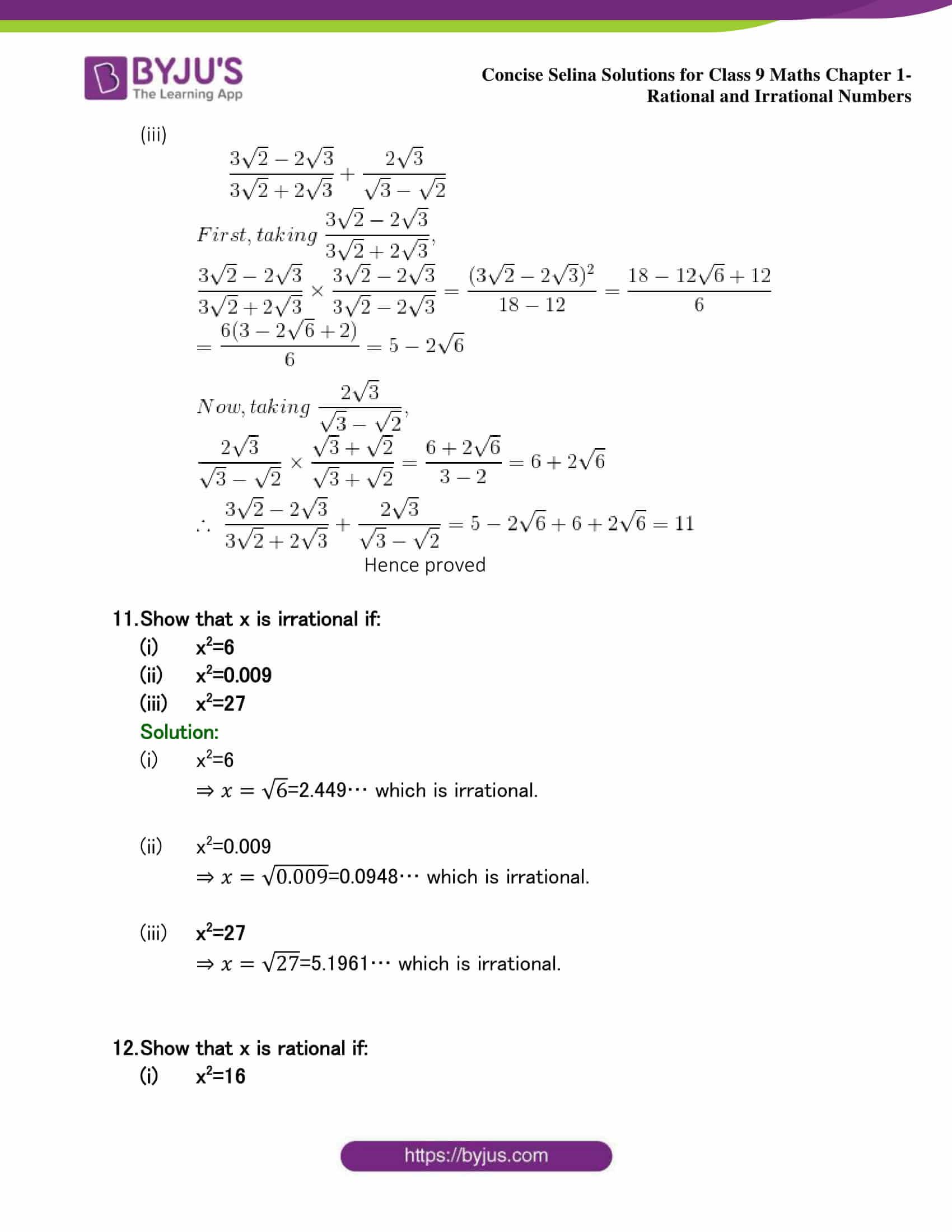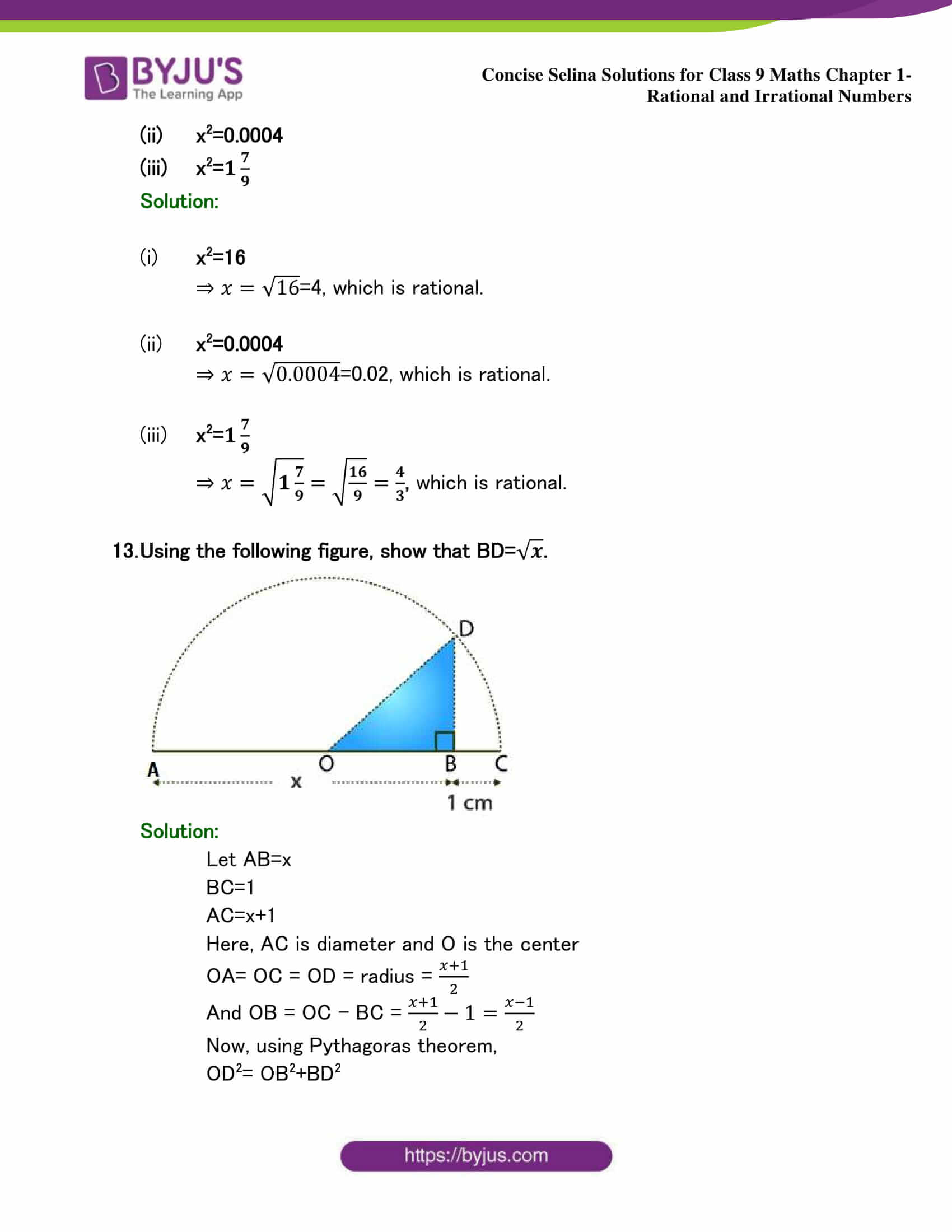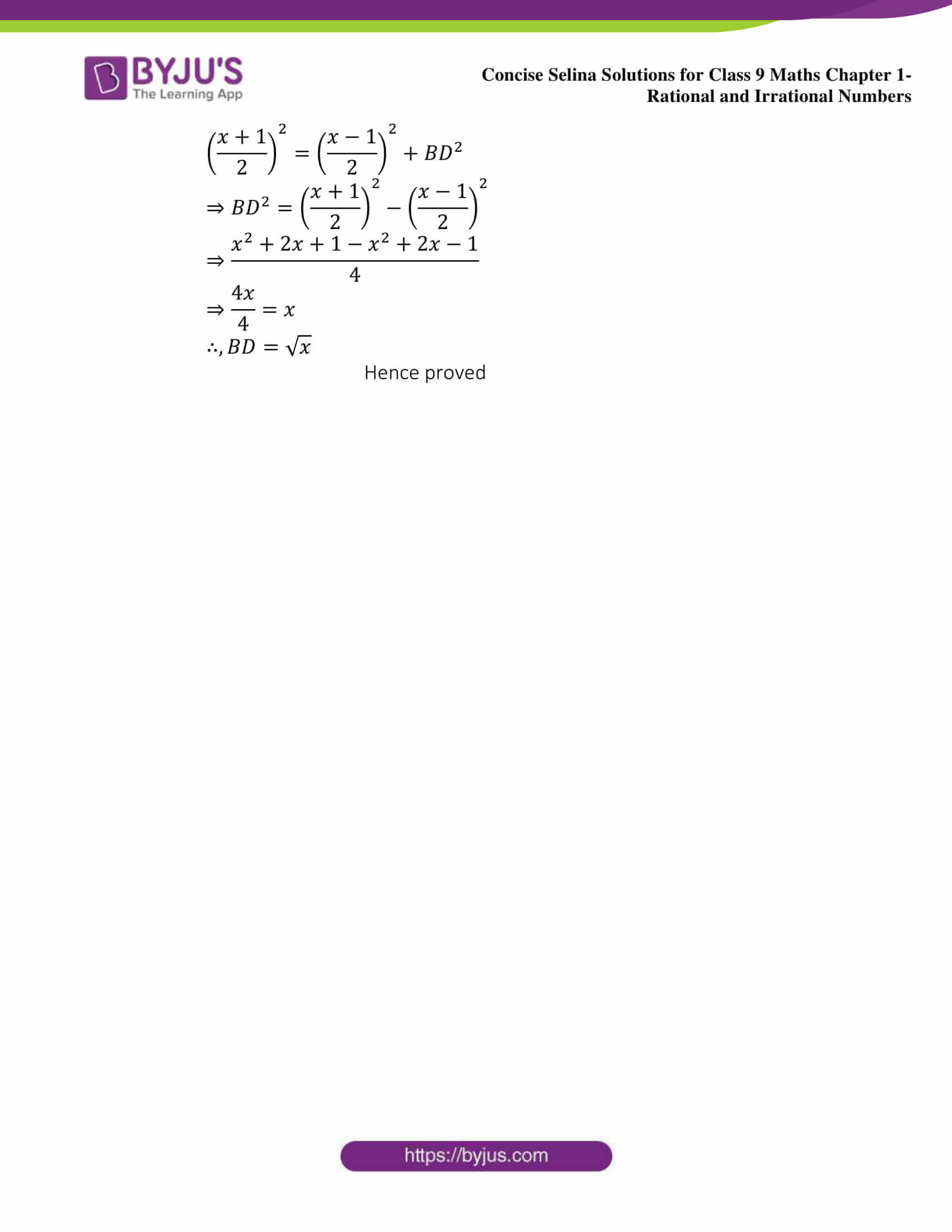## Selina Solutions for Class 9 Maths Chapter 1- Rational And Irrational Numbers

Chapter 1, Rational And Irrational Numbers, contains 4 exercises and the Selina Solutions given here contains the answers for all the questions present in these exercises. Let us have a look at some of the topics that are being discussed in this chapter.

1.1 Introduction

1.2 Rational Numbers

1.3 Properties of Rational Numbers

1.4 Decimal Representation of Rational Numbers

1.5 Irrational Numbers

1.6 Real Numbers